# Genetic Algorithms GA The most widely known type

• Slides: 97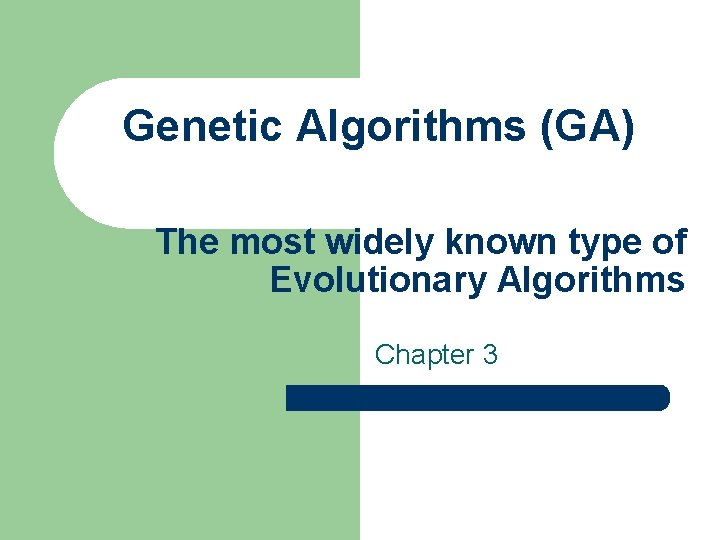Genetic Algorithms (GA) The most widely known type of Evolutionary Algorithms Chapter 3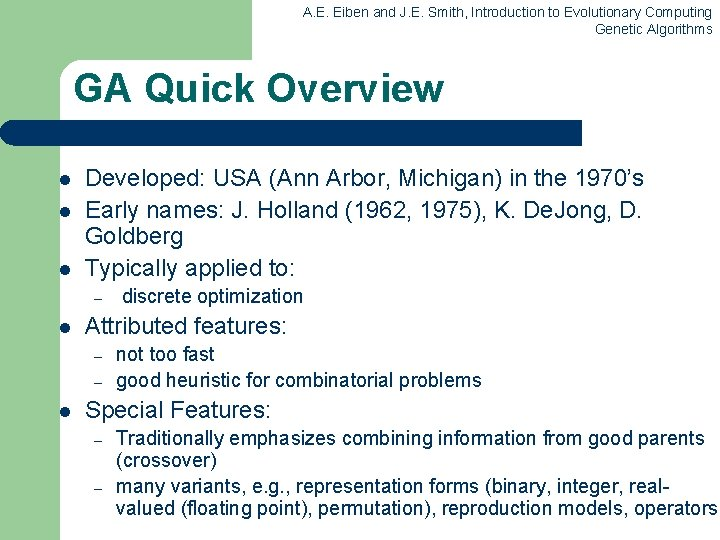A. E. Eiben and J. E. Smith, Introduction to Evolutionary Computing Genetic Algorithms GA Quick Overview l l l Developed: USA (Ann Arbor, Michigan) in the 1970’s Early names: J. Holland (1962, 1975), K. De. Jong, D. Goldberg Typically applied to: – l Attributed features: – – l discrete optimization not too fast good heuristic for combinatorial problems Special Features: – – Traditionally emphasizes combining information from good parents (crossover) many variants, e. g. , representation forms (binary, integer, realvalued (floating point), permutation), reproduction models, operators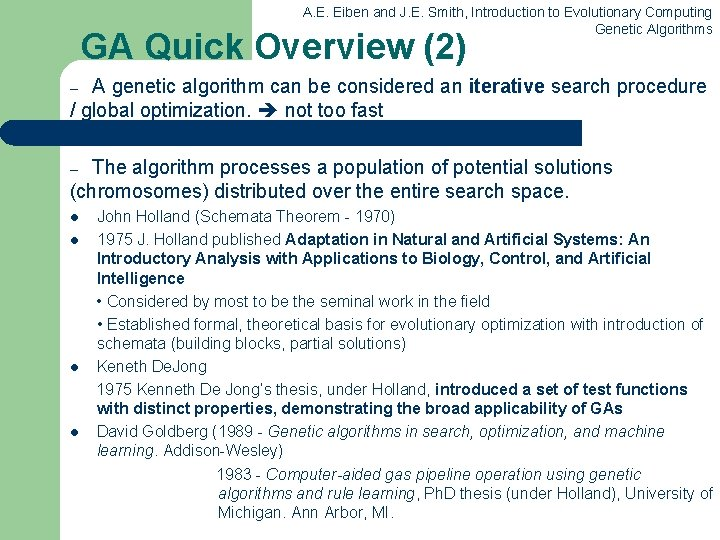A. E. Eiben and J. E. Smith, Introduction to Evolutionary Computing Genetic Algorithms GA Quick Overview (2) A genetic algorithm can be considered an iterative search procedure / global optimization. not too fast – The algorithm processes a population of potential solutions (chromosomes) distributed over the entire search space. – l l John Holland (Schemata Theorem - 1970) 1975 J. Holland published Adaptation in Natural and Artificial Systems: An Introductory Analysis with Applications to Biology, Control, and Artificial Intelligence • Considered by most to be the seminal work in the field • Established formal, theoretical basis for evolutionary optimization with introduction of schemata (building blocks, partial solutions) Keneth De. Jong 1975 Kenneth De Jong’s thesis, under Holland, introduced a set of test functions with distinct properties, demonstrating the broad applicability of GAs David Goldberg (1989 - Genetic algorithms in search, optimization, and machine learning. Addison-Wesley) 1983 - Computer-aided gas pipeline operation using genetic algorithms and rule learning, Ph. D thesis (under Holland), University of Michigan. Ann Arbor, MI.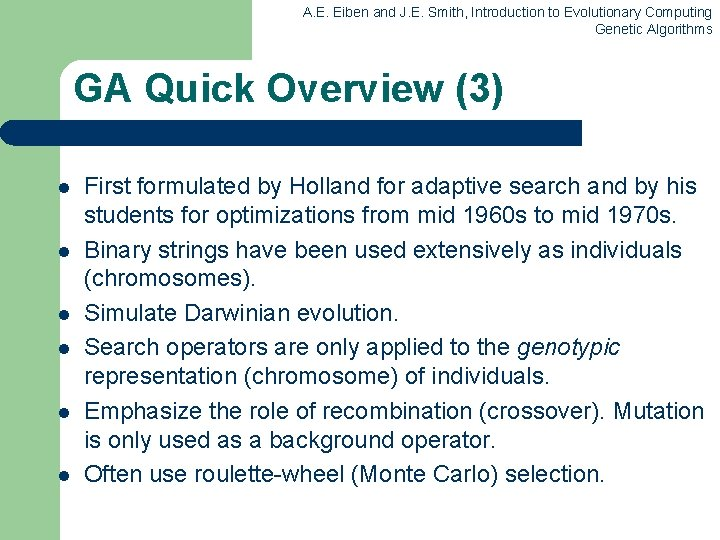A. E. Eiben and J. E. Smith, Introduction to Evolutionary Computing Genetic Algorithms GA Quick Overview (3) l l l First formulated by Holland for adaptive search and by his students for optimizations from mid 1960 s to mid 1970 s. Binary strings have been used extensively as individuals (chromosomes). Simulate Darwinian evolution. Search operators are only applied to the genotypic representation (chromosome) of individuals. Emphasize the role of recombination (crossover). Mutation is only used as a background operator. Often use roulette-wheel (Monte Carlo) selection.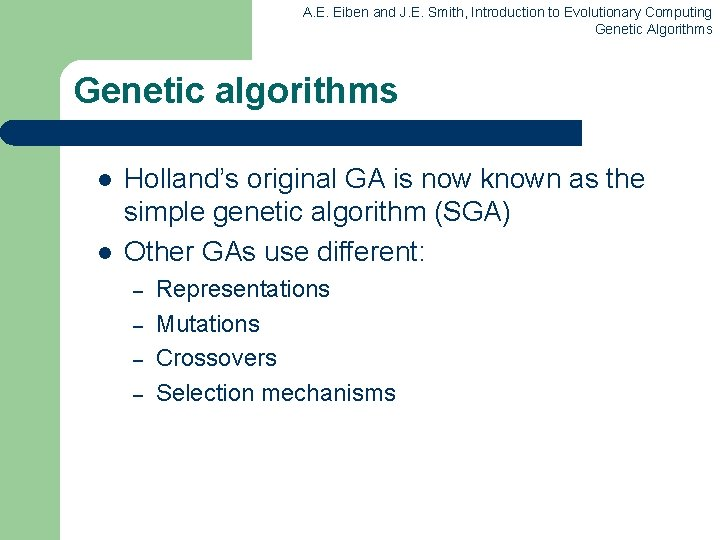A. E. Eiben and J. E. Smith, Introduction to Evolutionary Computing Genetic Algorithms Genetic algorithms l l Holland’s original GA is now known as the simple genetic algorithm (SGA) Other GAs use different: – – Representations Mutations Crossovers Selection mechanismsA. E. Eiben and J. E. Smith, Introduction to Evolutionary Computing Genetic Algorithms (pseudocod I) l An Example of a Genetic Algorithm: Procedure GA{ t = 0; Initialize P(t); Evaluate P(t); While (Not Done) { Parents(t) = Select_Parents(P(t)); Offspring(t) = Procreate(Parents(t)); Evaluate(Offspring(t)); P(t+1)= Select_Survivors(P(t), Offspring(t)); t = t + 1; }A. E. Eiben and J. E. Smith, Introduction to Evolutionary Computing Genetic Algorithms (pseudocod II) l l l l P 1: Choose a selection mechanism and a mechanism for scaling, if necessary. P 2: Set t = 0; and Initialize the population P(t). P 3: It evaluates the performance of each chromosome from P(t). It retains most powerful individual in the population P(t). P 4: Selection operator is applied n times (n – number of individuals). The selected chromosomes form an intermediate population P 1 (having also n members). In P 1, some chromosomes of P(t) can have more children (will appear more than once) and others have no copy. P 5: Apply crossover operator on the chromosomes of intermediate population P 1. The chromosomes obtained form a new population P 2. Deleted from the intermediate population P 1, the parents of chromosomes obtained by crossover. The remaining chromosomes from P 1 become members of the P 2 population. P 6: Apply mutation operator on the chromosomes of population P 2. The result is the new generation P(t+1). P 7: Set t = t + 1; If t≤N, where N is the maximum number of generation, then continues with step P 3. Otherwise STOP.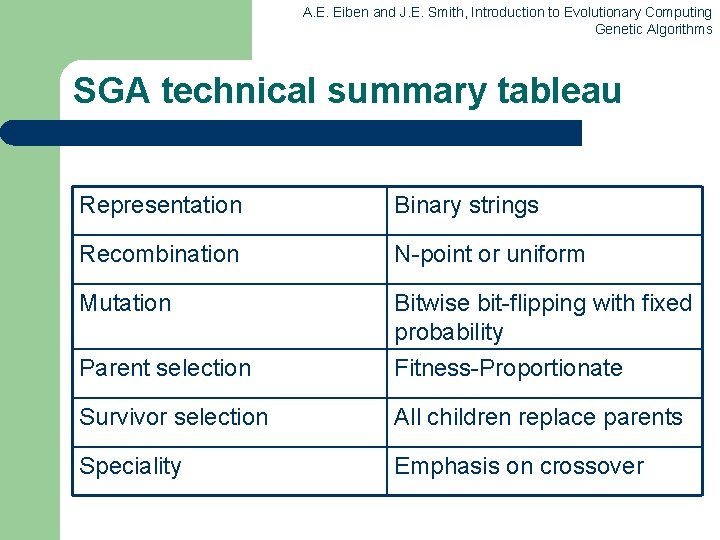A. E. Eiben and J. E. Smith, Introduction to Evolutionary Computing Genetic Algorithms SGA technical summary tableau Representation Binary strings Recombination N-point or uniform Mutation Parent selection Bitwise bit-flipping with fixed probability Fitness-Proportionate Survivor selection All children replace parents Speciality Emphasis on crossoverA. E. Eiben and J. E. Smith, Introduction to Evolutionary Computing Genetic Algorithms Representation - The most critical decision in any application, namely that of deciding how best to represent a candidate solution of the algorithm. Phenotype space Genotype space = {0, 1}L Encoding (representation) 10010001 10010010 01001 011101001 Decoding (inverse representation)A. E. Eiben and J. E. Smith, Introduction to Evolutionary Computing Genetic Algorithms SGA reproduction cycle 1. Select parents for the mating pool (size of mating pool = population size) 2. Shuffle the mating pool 3. For each consecutive pair apply crossover with probability pc , otherwise copy parents 4. For each offspring apply mutation (bit-flip with probability pm independently for each bit) 5. Replace the whole population with the resulting offspring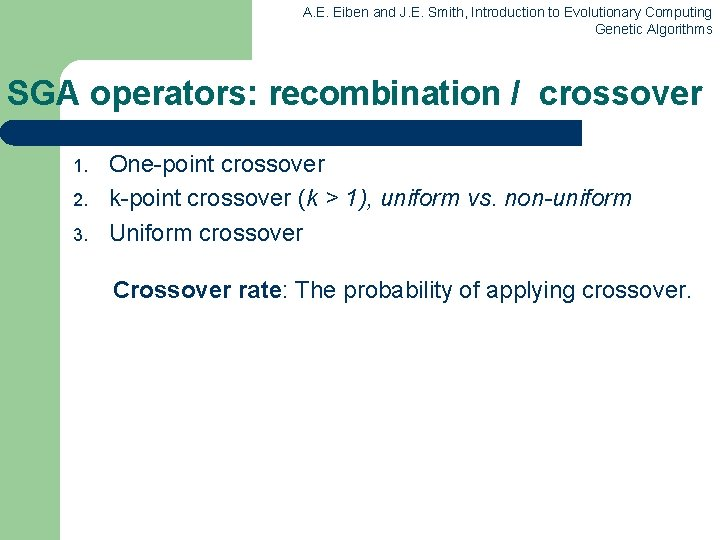A. E. Eiben and J. E. Smith, Introduction to Evolutionary Computing Genetic Algorithms SGA operators: recombination / crossover 1. 2. 3. One-point crossover k-point crossover (k > 1), uniform vs. non-uniform Uniform crossover Crossover rate: The probability of applying crossover.A. E. Eiben and J. E. Smith, Introduction to Evolutionary Computing Genetic Algorithms SGA operators: 1 -point crossover l l Choose a random point on the two parents Split parents at this crossover point Create children by exchanging tails Pc typically in range (0. 6, 0. 9)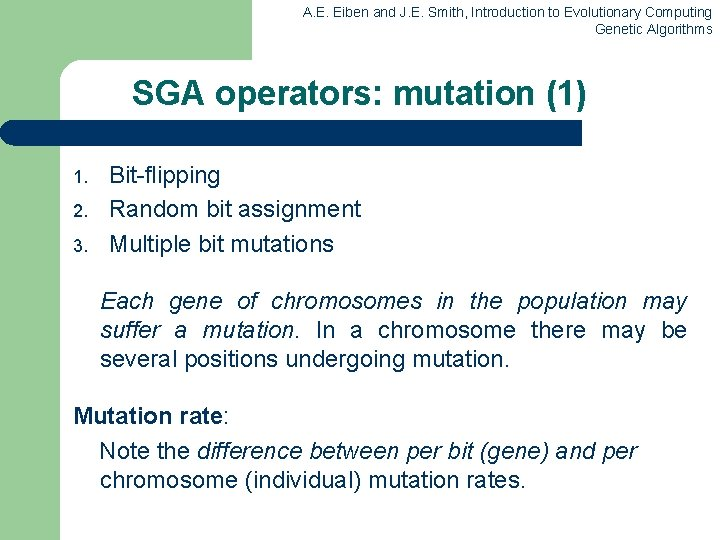A. E. Eiben and J. E. Smith, Introduction to Evolutionary Computing Genetic Algorithms SGA operators: mutation (1) 1. 2. 3. Bit-flipping Random bit assignment Multiple bit mutations Each gene of chromosomes in the population may suffer a mutation. In a chromosome there may be several positions undergoing mutation. Mutation rate: Note the difference between per bit (gene) and per chromosome (individual) mutation rates.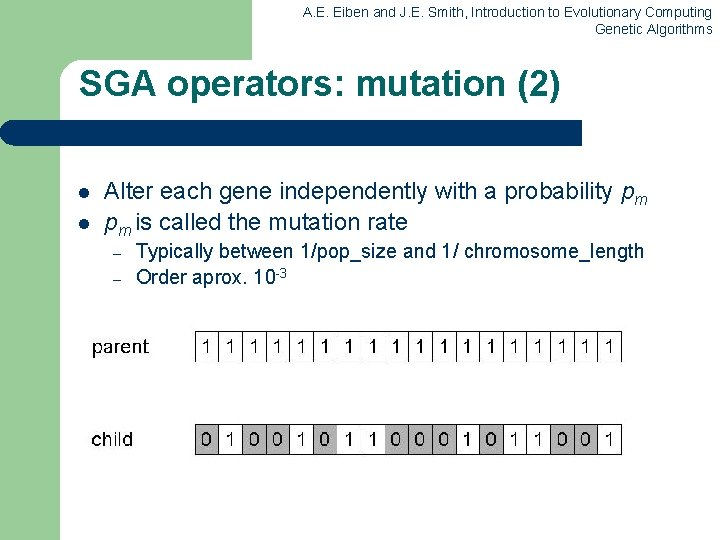A. E. Eiben and J. E. Smith, Introduction to Evolutionary Computing Genetic Algorithms SGA operators: mutation (2) l l Alter each gene independently with a probability pm pm is called the mutation rate – – Typically between 1/pop_size and 1/ chromosome_length Order aprox. 10 -3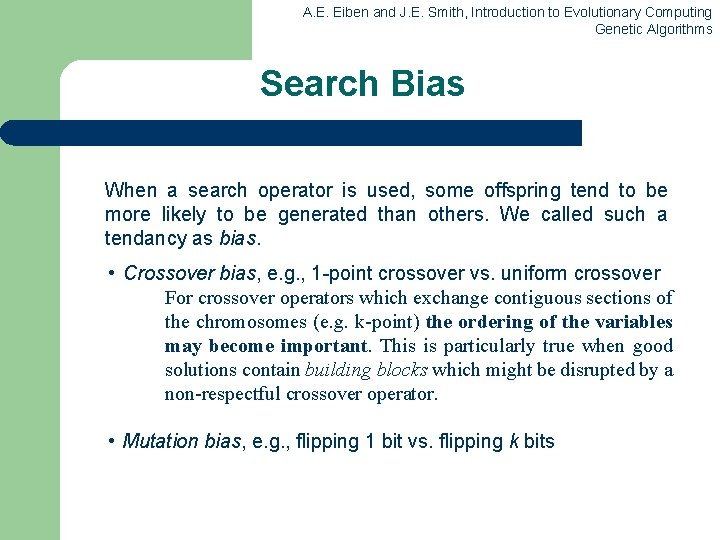A. E. Eiben and J. E. Smith, Introduction to Evolutionary Computing Genetic Algorithms Search Bias When a search operator is used, some offspring tend to be more likely to be generated than others. We called such a tendancy as bias. • Crossover bias, e. g. , 1 -point crossover vs. uniform crossover For crossover operators which exchange contiguous sections of the chromosomes (e. g. k-point) the ordering of the variables may become important. This is particularly true when good solutions contain building blocks which might be disrupted by a non-respectful crossover operator. • Mutation bias, e. g. , flipping 1 bit vs. flipping k bitsA. E. Eiben and J. E. Smith, Introduction to Evolutionary Computing Genetic Algorithms SGA operators: Selection l Main idea: better individuals get higher chance – Chances proportional to fitness – Implementation: roulette wheel technique l Assign to each individual a part of the roulette wheel l Spin the wheel n times to select n individuals 1/6 = 17% A 3/6 = 50% B C 2/6 = 33% fitness(A) = 3 fitness(B) = 1 fitness(C) = 2A. E. Eiben and J. E. Smith, Introduction to Evolutionary Computing Genetic Algorithms: Proportionate Selection l l In Proportionate Selection, individuals are assigned a probability of being selected based on their fitness: – pi = fi / fj – Where pi is the probability that individual i will be selected, – fi is the fitness of individual i, and – fj represents the sum of all the fitnesses of the individuals with the population. This type of selection is similar to using a roulette wheel where the fitness of an individual is represented as proportionate slice of wheel. The wheel is then spun and the slice underneath the wheel when it stops determine which individual becomes a parent.A. E. Eiben and J. E. Smith, Introduction to Evolutionary Computing Genetic Algorithms: Roulette wheel selection scheme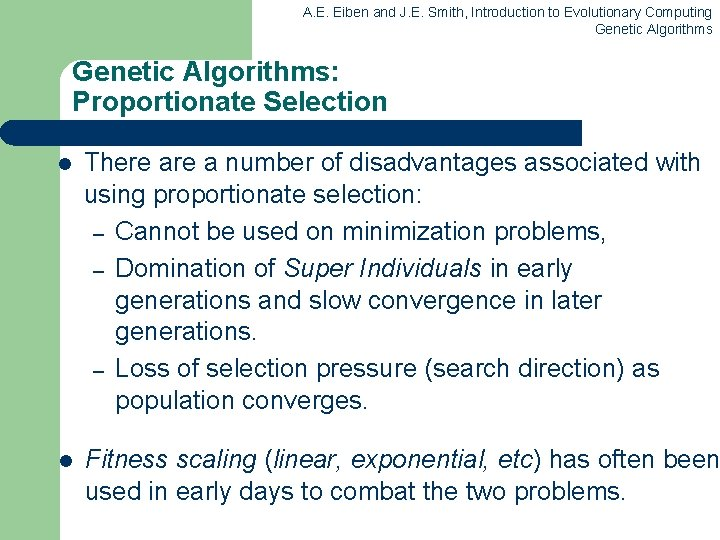A. E. Eiben and J. E. Smith, Introduction to Evolutionary Computing Genetic Algorithms: Proportionate Selection l There a number of disadvantages associated with using proportionate selection: – Cannot be used on minimization problems, – Domination of Super Individuals in early generations and slow convergence in later generations. – Loss of selection pressure (search direction) as population converges. l Fitness scaling (linear, exponential, etc) has often been used in early days to combat the two problems.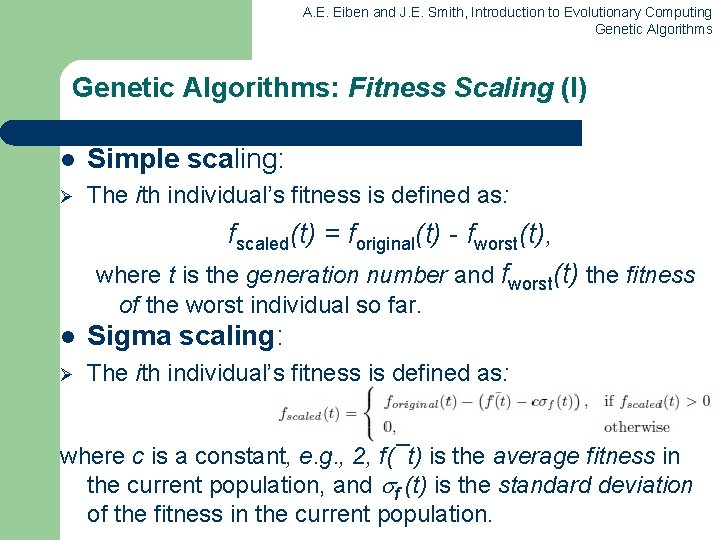A. E. Eiben and J. E. Smith, Introduction to Evolutionary Computing Genetic Algorithms: Fitness Scaling (I) l Simple scaling: Ø The ith individual’s fitness is defined as: fscaled(t) = foriginal(t) - fworst(t), where t is the generation number and fworst(t) the fitness of the worst individual so far. l Sigma scaling: Ø The ith individual’s fitness is defined as: where c is a constant, e. g. , 2, f(¯t) is the average fitness in the current population, and f (t) is the standard deviation of the fitness in the current population.A. E. Eiben and J. E. Smith, Introduction to Evolutionary Computing Genetic Algorithms: Fitness Scaling (II) l Power scaling: Ø The ith individual’s fitness is defined as: fscaled(t) = (foriginal(t))k, where k > 0. l Exponential scaling: Ø The ith individual’s fitness is defined as: fscaled(t) = exp(foriginal(t)/T ) where T > 0 is the temperature, approaching zero.A. E. Eiben and J. E. Smith, Introduction to Evolutionary Computing Genetic Algorithms An example after Goldberg ‘ 89 (1) l l Simple problem: max x 2 over {0, 1, …, 31} GA approach: – – – l Representation: binary code, e. g. 01101 13 Population size: 4 1 -point xover, bitwise mutation Roulette wheel selection Random initialisation We show one generational cycle done by hand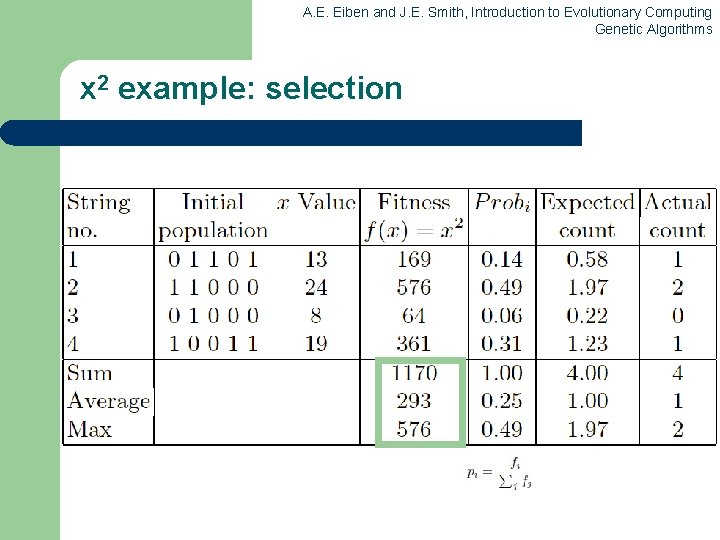A. E. Eiben and J. E. Smith, Introduction to Evolutionary Computing Genetic Algorithms x 2 example: selection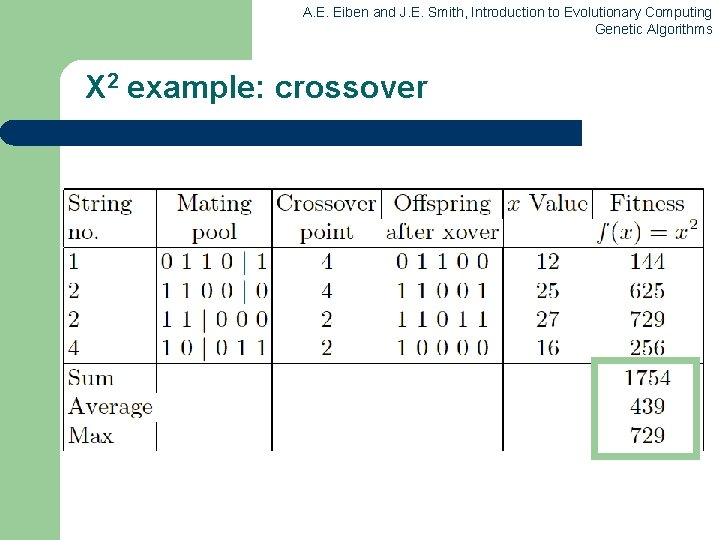A. E. Eiben and J. E. Smith, Introduction to Evolutionary Computing Genetic Algorithms X 2 example: crossoverA. E. Eiben and J. E. Smith, Introduction to Evolutionary Computing Genetic Algorithms X 2 example: mutation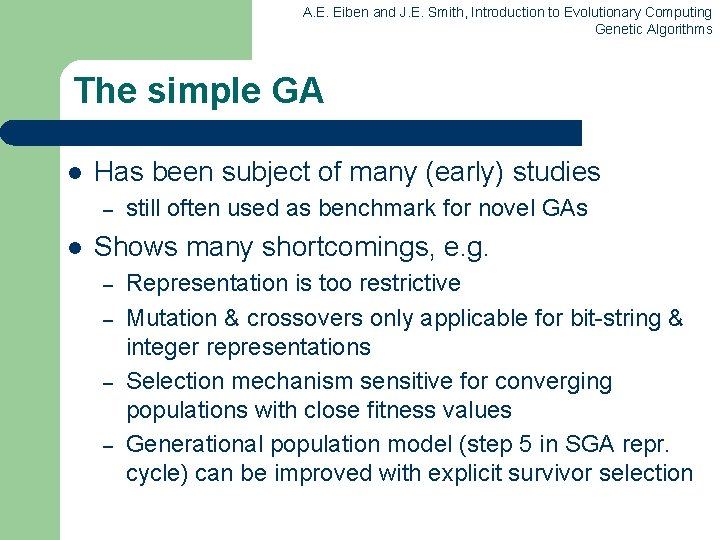A. E. Eiben and J. E. Smith, Introduction to Evolutionary Computing Genetic Algorithms The simple GA l Has been subject of many (early) studies – l still often used as benchmark for novel GAs Shows many shortcomings, e. g. – – Representation is too restrictive Mutation & crossovers only applicable for bit-string & integer representations Selection mechanism sensitive for converging populations with close fitness values Generational population model (step 5 in SGA repr. cycle) can be improved with explicit survivor selection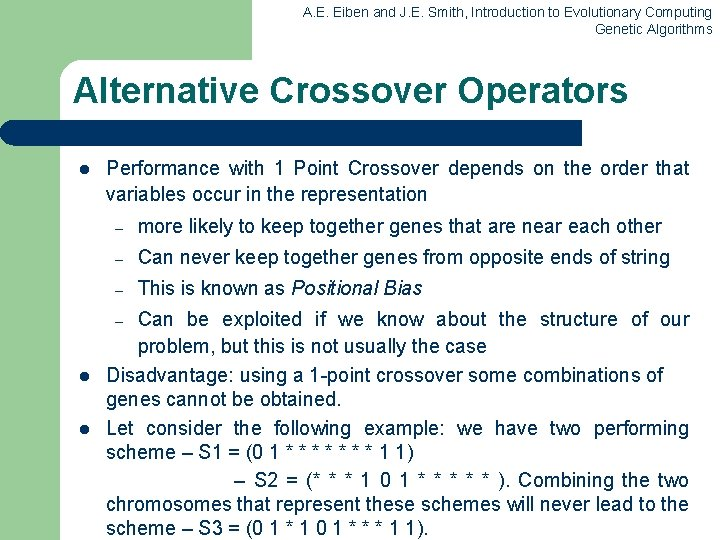A. E. Eiben and J. E. Smith, Introduction to Evolutionary Computing Genetic Algorithms Alternative Crossover Operators l Performance with 1 Point Crossover depends on the order that variables occur in the representation – more likely to keep together genes that are near each other – Can never keep together genes from opposite ends of string – This is known as Positional Bias Can be exploited if we know about the structure of our problem, but this is not usually the case Disadvantage: using a 1 -point crossover some combinations of genes cannot be obtained. Let consider the following example: we have two performing scheme – S 1 = (0 1 * * * * 1 1) – S 2 = (* * * 1 0 1 * * * ). Combining the two chromosomes that represent these schemes will never lead to the scheme – S 3 = (0 1 * 1 0 1 * * * 1 1). – l lA. E. Eiben and J. E. Smith, Introduction to Evolutionary Computing Genetic Algorithms n-point crossover l l Choose n random crossover points Split along those points Glue parts, alternating between parents Generalisation of 1 point (still some positional bias)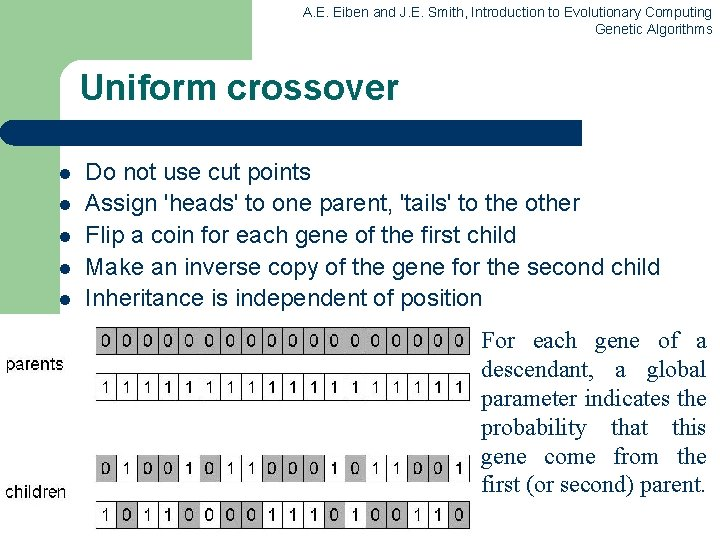A. E. Eiben and J. E. Smith, Introduction to Evolutionary Computing Genetic Algorithms Uniform crossover l l l Do not use cut points Assign 'heads' to one parent, 'tails' to the other Flip a coin for each gene of the first child Make an inverse copy of the gene for the second child Inheritance is independent of position For each gene of a descendant, a global parameter indicates the probability that this gene come from the first (or second) parent.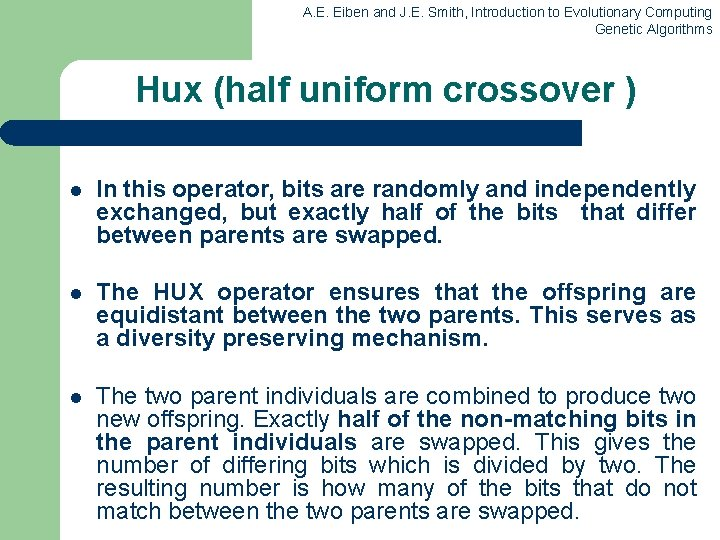A. E. Eiben and J. E. Smith, Introduction to Evolutionary Computing Genetic Algorithms Hux (half uniform crossover ) l In this operator, bits are randomly and independently exchanged, but exactly half of the bits that differ between parents are swapped. l The HUX operator ensures that the offspring are equidistant between the two parents. This serves as a diversity preserving mechanism. l The two parent individuals are combined to produce two new offspring. Exactly half of the non-matching bits in the parent individuals are swapped. This gives the number of differing bits which is divided by two. The resulting number is how many of the bits that do not match between the two parents are swapped.A. E. Eiben and J. E. Smith, Introduction to Evolutionary Computing Genetic Algorithms ExampleA. E. Eiben and J. E. Smith, Introduction to Evolutionary Computing Genetic Algorithms Alternative Mutation Operators Through mutation are introduced in the population individuals which could not be generated by other mechanisms. • • • Strong Mutation Weak Mutation Single chromosome (individual) mutation Not uniform mutation Adaptive not-uniform mutation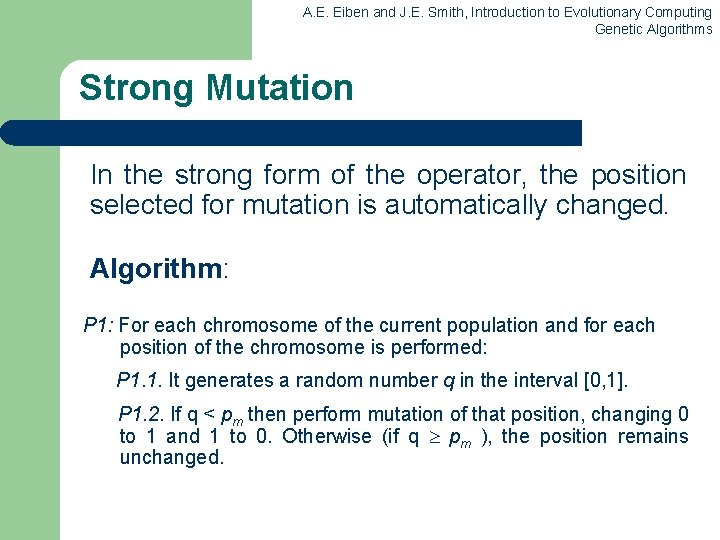A. E. Eiben and J. E. Smith, Introduction to Evolutionary Computing Genetic Algorithms Strong Mutation In the strong form of the operator, the position selected for mutation is automatically changed. Algorithm: P 1: For each chromosome of the current population and for each position of the chromosome is performed: P 1. 1. It generates a random number q in the interval [0, 1]. P 1. 2. If q < pm then perform mutation of that position, changing 0 to 1 and 1 to 0. Otherwise (if q pm ), the position remains unchanged.A. E. Eiben and J. E. Smith, Introduction to Evolutionary Computing Genetic Algorithms Weak Mutation In the weak form of the operator, the position selected for mutation is NOT automatically changed. New value of the position which meets the condition of mutation, is generated randomly. Algorithm: P 1: For each chromosome of the current population and for each position of the chromosome is performed: P 1. 1. It generates a random number q in the interval [0, 1]. P 1. 2’. If q < pm then randomly choose one of the values 0 or 1. Current position is assigned as the value selected. Otherwise (if q pm ), the position remains unchanged.A. E. Eiben and J. E. Smith, Introduction to Evolutionary Computing Genetic Algorithms Single chromosome (individual) Mutation In this version, each application of the operator affects a gene (or possibly more) of a chromosome (which was selected for mutation). l You can use both the weak and the strong version of the mutation operator. l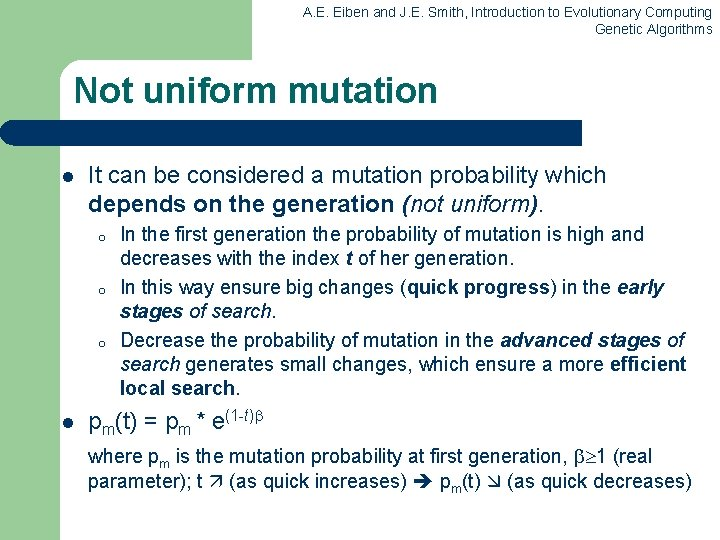A. E. Eiben and J. E. Smith, Introduction to Evolutionary Computing Genetic Algorithms Not uniform mutation l It can be considered a mutation probability which depends on the generation (not uniform). o o o l In the first generation the probability of mutation is high and decreases with the index t of her generation. In this way ensure big changes (quick progress) in the early stages of search. Decrease the probability of mutation in the advanced stages of search generates small changes, which ensure a more efficient local search. pm(t) = pm * e(1 -t) where pm is the mutation probability at first generation, 1 (real parameter); t (as quick increases) pm(t) (as quick decreases)A. E. Eiben and J. E. Smith, Introduction to Evolutionary Computing Genetic Algorithms Adaptive not-uniform mutation l The mutation probability depends on the position (gene rank) in chromosome and generation (t). o o o l In the first generations perform a global search In the last part of the process it performs a local search As the generation index (t) increases the probability of mutation of the first genes from chromosome to decrease, and of the last genes to grow. Example: o o Let’s consider the search space being . The chromosomes binary encode the real values. If a gene is positioned at the beginning of a chromosome, then its mutation it causes a significant change of the chromosome. Elements of search space corresponding to the two chromosomes (at the beginning and that containing gene changed) will be very different. If mutation occurs at the end of the chromosome will result in a much smaller change.A. E. Eiben and J. E. Smith, Introduction to Evolutionary Computing Genetic Algorithms Crossover OR mutation? l l l Decade long debate: which one is better / necessary / mainbackground Answer (at least, rather wide agreement): – it depends on the problem, but – in general, it is good to have both – both have another role – mutation-only-EA is possible, xover-only-EA would not work Achieving a balance between information exploitation and by the state-space exploration to obtain new better solutions, is typical of all powerful optimization methods. If the solutions obtained are exploited too much, then reaches a premature convergence. On the other hand, if too much emphasis on exploration, it is possible that the information already obtained is not used properly. Search time grows and approaches that of random search methods.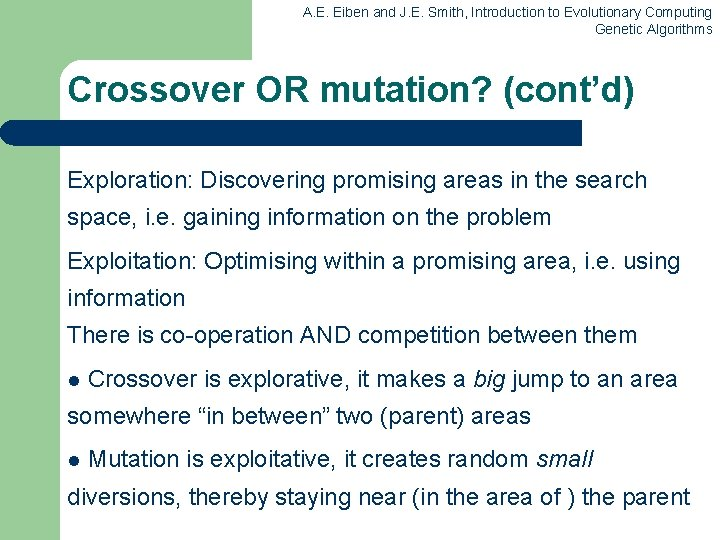A. E. Eiben and J. E. Smith, Introduction to Evolutionary Computing Genetic Algorithms Crossover OR mutation? (cont’d) Exploration: Discovering promising areas in the search space, i. e. gaining information on the problem Exploitation: Optimising within a promising area, i. e. using information There is co-operation AND competition between them l Crossover is explorative, it makes a big jump to an area somewhere “in between” two (parent) areas l Mutation is exploitative, it creates random small diversions, thereby staying near (in the area of ) the parentA. E. Eiben and J. E. Smith, Introduction to Evolutionary Computing Genetic Algorithms Crossover OR mutation? (cont’d) l Only crossover can combine information from two parents l Only mutation can introduce new information (alleles) l Crossover does not change the allele frequencies of the population (thought experiment: 50% 0’s on first bit in the population, ? % after performing n crossovers) l To hit the optimum you often need a ‘lucky’ mutation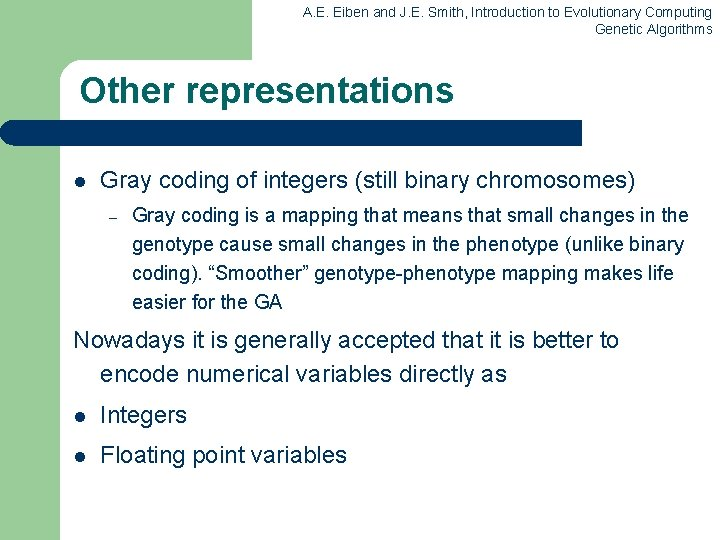A. E. Eiben and J. E. Smith, Introduction to Evolutionary Computing Genetic Algorithms Other representations l Gray coding of integers (still binary chromosomes) – Gray coding is a mapping that means that small changes in the genotype cause small changes in the phenotype (unlike binary coding). “Smoother” genotype-phenotype mapping makes life easier for the GA Nowadays it is generally accepted that it is better to encode numerical variables directly as l Integers l Floating point variables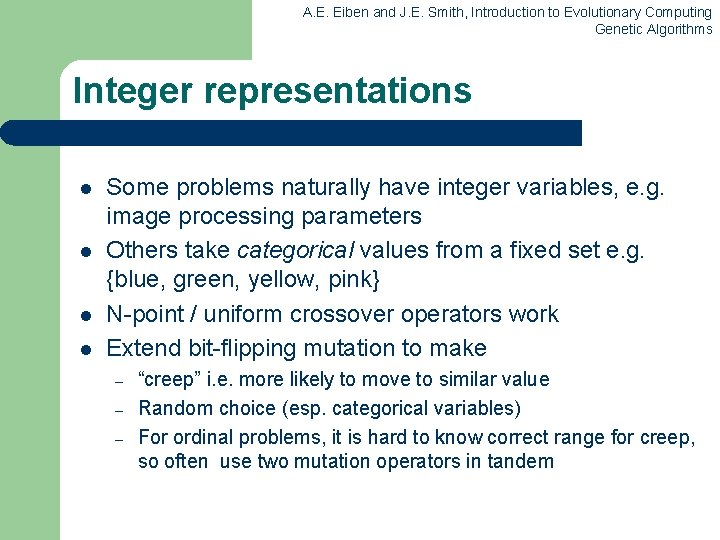A. E. Eiben and J. E. Smith, Introduction to Evolutionary Computing Genetic Algorithms Integer representations l l Some problems naturally have integer variables, e. g. image processing parameters Others take categorical values from a fixed set e. g. {blue, green, yellow, pink} N-point / uniform crossover operators work Extend bit-flipping mutation to make – – – “creep” i. e. more likely to move to similar value Random choice (esp. categorical variables) For ordinal problems, it is hard to know correct range for creep, so often use two mutation operators in tandem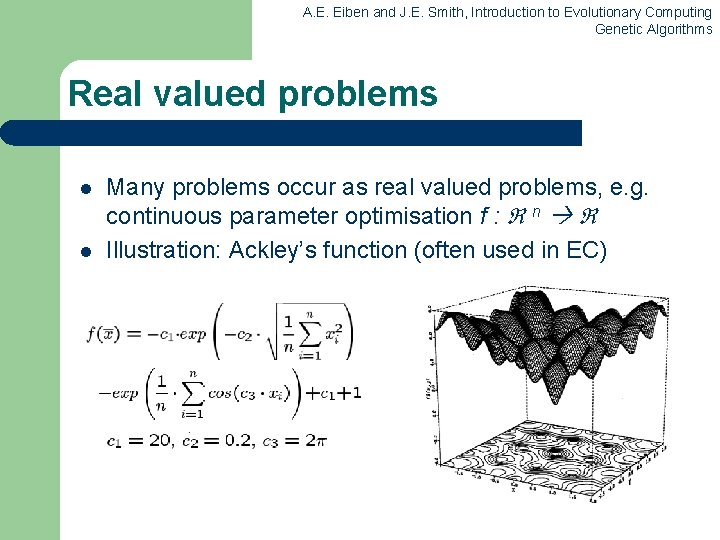A. E. Eiben and J. E. Smith, Introduction to Evolutionary Computing Genetic Algorithms Real valued problems l l Many problems occur as real valued problems, e. g. continuous parameter optimisation f : n Illustration: Ackley’s function (often used in EC)A. E. Eiben and J. E. Smith, Introduction to Evolutionary Computing Genetic Algorithms Using a GA for Neural Network training in order to increase prediction accuracy (PA) l l l S-a stabilit o populaţie de cromozomi suficient de largă (50) pentru a asigura variabilitatea populaţiei Fiecare cromozom conţine un număr de gene, acestea reprezentând de fapt o pondere din cadrul reţelei neurale. Genele aparţinând populaţiei iniţiale de cromozomi sunt iniţializate cu valori aleatoare în intervalul [-1, 1]. Algoritmul genetic se desfăşoară după scenariul descris în partea teoretică a lucrării: – – – pentru un număr de generaţii stabilit se repetă paşii următori (aprox. 40) sunt evaluaţi toţi cromozomii (fitness = PA – prediction accuracy) este aplicat operatorul de încrucişare (Crossover) este aplicat operatorul de mutaţie (Mutation) cel mai performant cromozom din generaţia finală va conţine prin genele sale ponderile reţelei antrenate static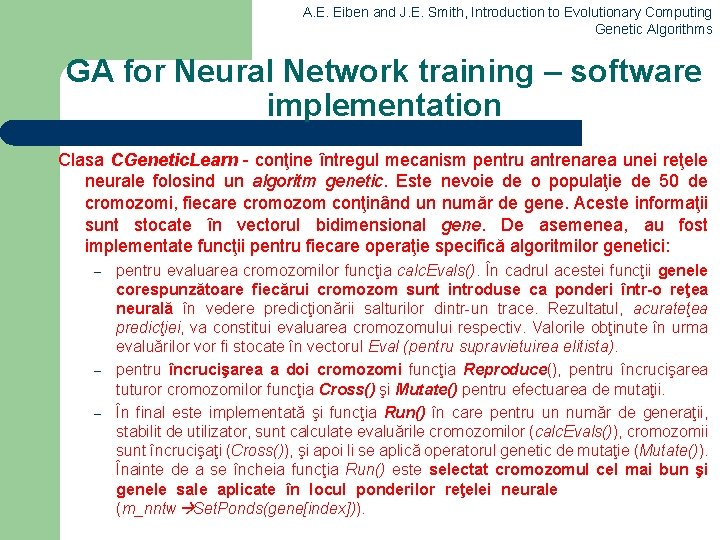A. E. Eiben and J. E. Smith, Introduction to Evolutionary Computing Genetic Algorithms GA for Neural Network training – software implementation Clasa CGenetic. Learn - conţine întregul mecanism pentru antrenarea unei reţele neurale folosind un algoritm genetic. Este nevoie de o populaţie de 50 de cromozomi, fiecare cromozom conţinând un număr de gene. Aceste informaţii sunt stocate în vectorul bidimensional gene. De asemenea, au fost implementate funcţii pentru fiecare operaţie specifică algoritmilor genetici: – – – pentru evaluarea cromozomilor funcţia calc. Evals(). În cadrul acestei funcţii genele corespunzătoare fiecărui cromozom sunt introduse ca ponderi într-o reţea neurală în vedere predicţionării salturilor dintr-un trace. Rezultatul, acurateţea predicţiei, va constitui evaluarea cromozomului respectiv. Valorile obţinute în urma evaluărilor vor fi stocate în vectorul Eval (pentru supravietuirea elitista). pentru încrucişarea a doi cromozomi funcţia Reproduce(), pentru încrucişarea tuturor cromozomilor funcţia Cross() şi Mutate() pentru efectuarea de mutaţii. În final este implementată şi funcţia Run() în care pentru un număr de generaţii, stabilit de utilizator, sunt calculate evaluările cromozomilor (calc. Evals()), cromozomii sunt încrucişaţi (Cross()), şi apoi li se aplică operatorul genetic de mutaţie (Mutate()). Înainte de a se încheia funcţia Run() este selectat cromozomul cel mai bun şi genele sale aplicate în locul ponderilor reţelei neurale (m_nntw Set. Ponds(gene[index])).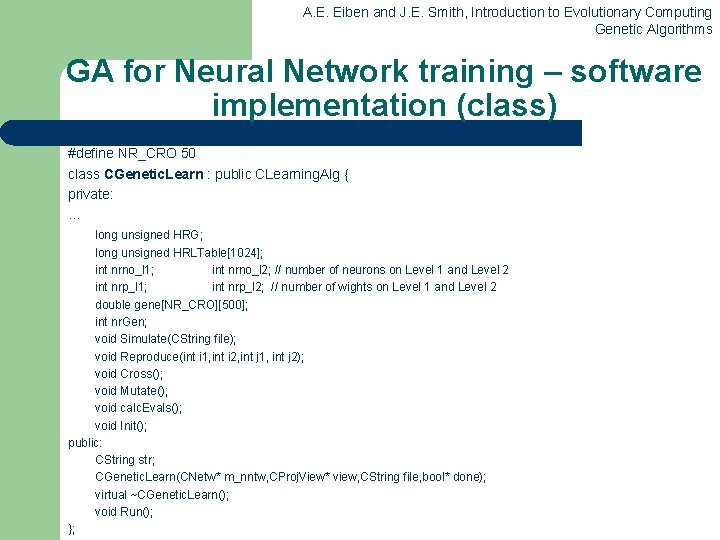A. E. Eiben and J. E. Smith, Introduction to Evolutionary Computing Genetic Algorithms GA for Neural Network training – software implementation (class) #define NR_CRO 50 class CGenetic. Learn : public CLearning. Alg { private: . . . long unsigned HRG; long unsigned HRLTable; int nrno_l 1; int nrno_l 2; // number of neurons on Level 1 and Level 2 int nrp_l 1; int nrp_l 2; // number of wights on Level 1 and Level 2 double gene[NR_CRO]; int nr. Gen; void Simulate(CString file); void Reproduce(int i 1, int i 2, int j 1, int j 2); void Cross(); void Mutate(); void calc. Evals(); void Init(); public: CString str; CGenetic. Learn(CNetw* m_nntw, CProj. View* view, CString file, bool* done); virtual ~CGenetic. Learn(); void Run(); };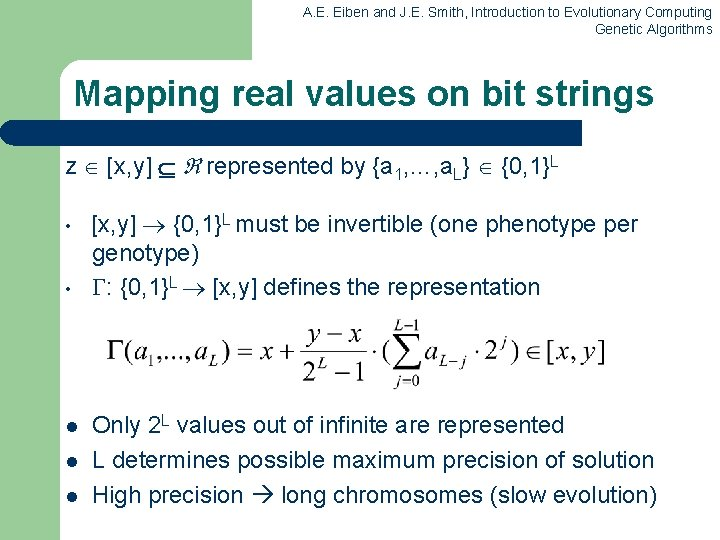A. E. Eiben and J. E. Smith, Introduction to Evolutionary Computing Genetic Algorithms Mapping real values on bit strings z [x, y] represented by {a 1, …, a. L} {0, 1}L • • l l l [x, y] {0, 1}L must be invertible (one phenotype per genotype) : {0, 1}L [x, y] defines the representation Only 2 L values out of infinite are represented L determines possible maximum precision of solution High precision long chromosomes (slow evolution)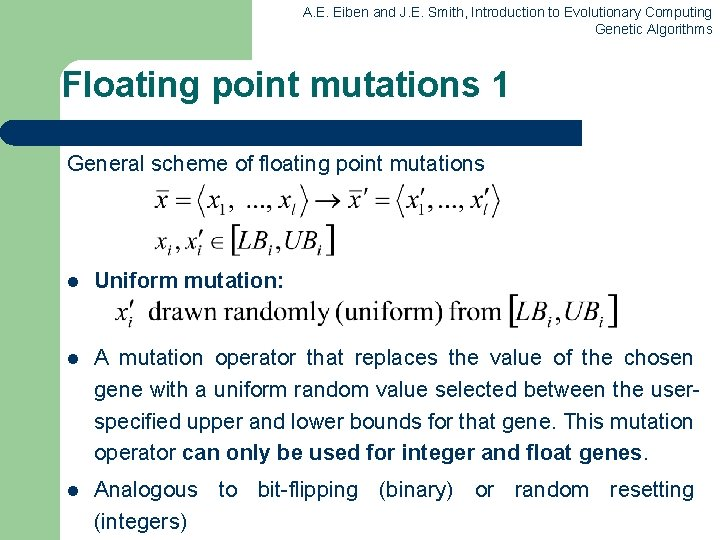A. E. Eiben and J. E. Smith, Introduction to Evolutionary Computing Genetic Algorithms Floating point mutations 1 General scheme of floating point mutations l Uniform mutation: l A mutation operator that replaces the value of the chosen gene with a uniform random value selected between the userspecified upper and lower bounds for that gene. This mutation operator can only be used for integer and float genes. l Analogous to bit-flipping (binary) or random resetting (integers)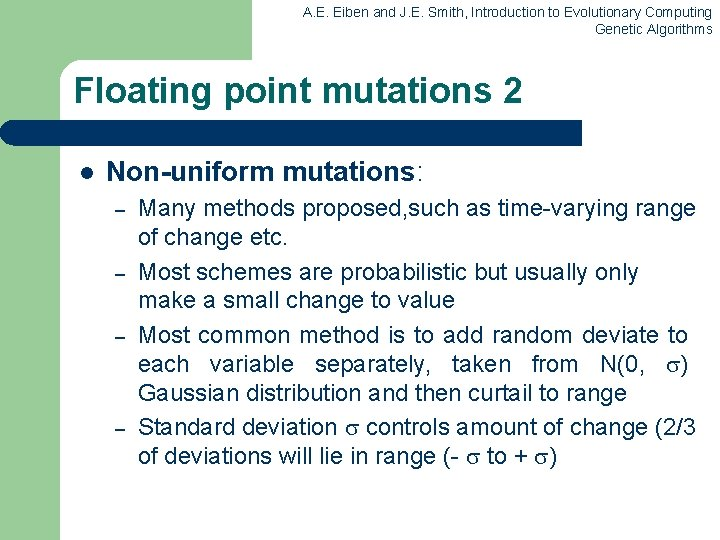A. E. Eiben and J. E. Smith, Introduction to Evolutionary Computing Genetic Algorithms Floating point mutations 2 l Non-uniform mutations: – – Many methods proposed, such as time-varying range of change etc. Most schemes are probabilistic but usually only make a small change to value Most common method is to add random deviate to each variable separately, taken from N(0, ) Gaussian distribution and then curtail to range Standard deviation controls amount of change (2/3 of deviations will lie in range (- to + )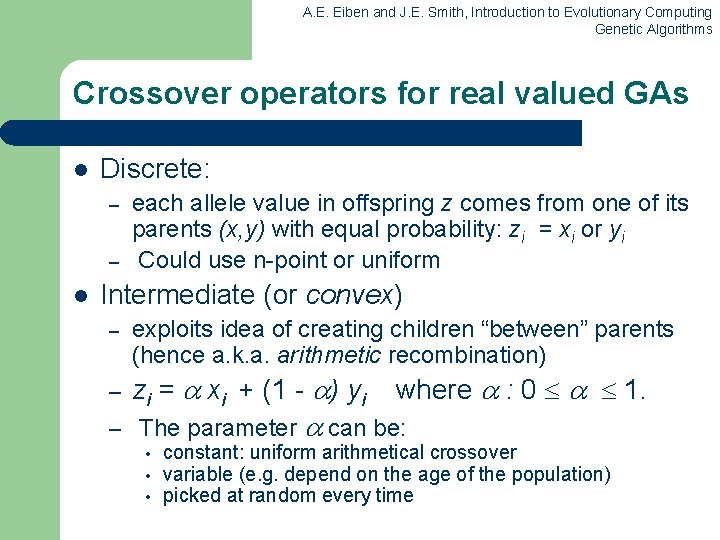A. E. Eiben and J. E. Smith, Introduction to Evolutionary Computing Genetic Algorithms Crossover operators for real valued GAs l Discrete: – – l each allele value in offspring z comes from one of its parents (x, y) with equal probability: zi = xi or yi Could use n-point or uniform Intermediate (or convex) – – exploits idea of creating children “between” parents (hence a. k. a. arithmetic recombination) zi = xi + (1 - ) yi – The parameter • • • where : 0 1. can be: constant: uniform arithmetical crossover variable (e. g. depend on the age of the population) picked at random every time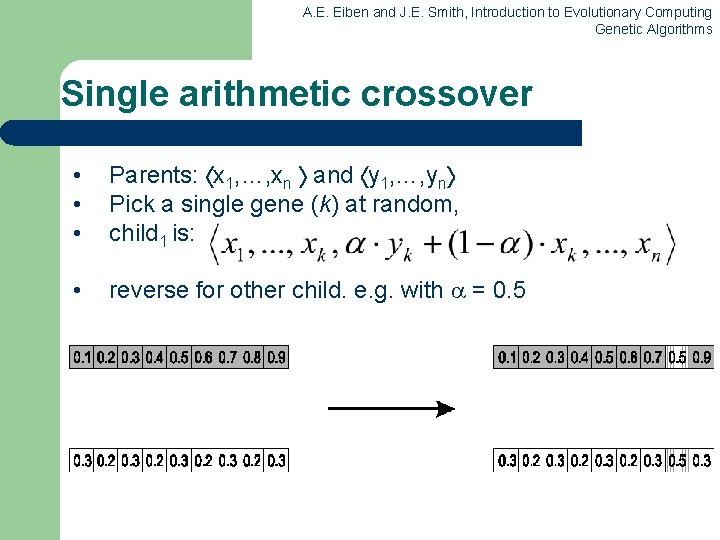A. E. Eiben and J. E. Smith, Introduction to Evolutionary Computing Genetic Algorithms Single arithmetic crossover • • • Parents: x 1, …, xn and y 1, …, yn Pick a single gene (k) at random, child 1 is: • reverse for other child. e. g. with = 0. 5A. E. Eiben and J. E. Smith, Introduction to Evolutionary Computing Genetic Algorithms Simple arithmetic crossover • • • Parents: x 1, …, xn and y 1, …, yn Pick random gene (k) after this point mix values child 1 is: • reverse for other child. e. g. with = 0. 5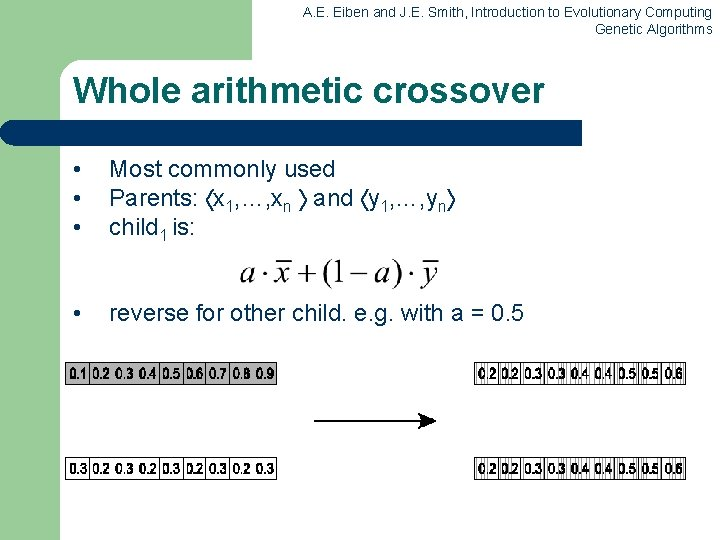A. E. Eiben and J. E. Smith, Introduction to Evolutionary Computing Genetic Algorithms Whole arithmetic crossover • • • Most commonly used Parents: x 1, …, xn and y 1, …, yn child 1 is: • reverse for other child. e. g. with a = 0. 5A. E. Eiben and J. E. Smith, Introduction to Evolutionary Computing Genetic Algorithms Permutation Representations l l Ordering/sequencing problems form a special type Task is (or can be solved by) arranging some objects in a certain order – – l Example: sort algorithm: important thing is which elements occur before others (order) Example: Travelling Salesman Problem (TSP) : important thing is which elements occur next to each other (adjacency) These problems are generally expressed as a permutation: – if there are n variables then the representation is as a list of n integers, each of which occurs exactly onceA. E. Eiben and J. E. Smith, Introduction to Evolutionary Computing Genetic Algorithms Permutation representation: TSP example l l l Problem: • Given n cities • Find a complete tour with minimal length Encoding: • Label the cities 1, 2, … , n • One complete tour is one permutation (e. g. for n =4 [1, 2, 3, 4], [3, 4, 2, 1] are OK) Search space is BIG: for 30 cities there are 30! 1032 possible toursA. E. Eiben and J. E. Smith, Introduction to Evolutionary Computing Genetic Algorithms Mutation operators for permutations l Normal mutation operators lead to inadmissible solutions – – l l e. g. bit-wise mutation : let gene i have value j changing to some other value k would mean that k occurred twice and j no longer occurred Therefore must change at least two values Mutation parameter now reflects the probability that some operator is applied once to the whole string, rather than individually in each positionA. E. Eiben and J. E. Smith, Introduction to Evolutionary Computing Genetic Algorithms Insert Mutation for permutations l l l Pick two allele values at random Move the second to follow the first, shifting the rest along to accommodate Note that this preserves most of the order and the adjacency information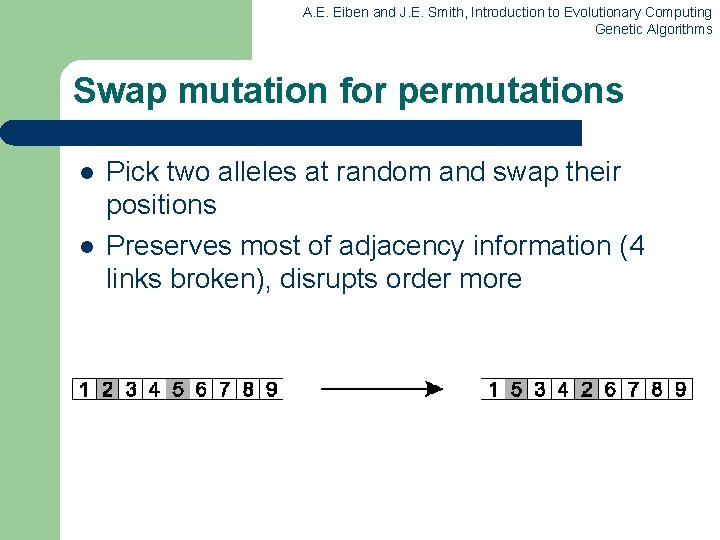A. E. Eiben and J. E. Smith, Introduction to Evolutionary Computing Genetic Algorithms Swap mutation for permutations l l Pick two alleles at random and swap their positions Preserves most of adjacency information (4 links broken), disrupts order moreA. E. Eiben and J. E. Smith, Introduction to Evolutionary Computing Genetic Algorithms Inversion mutation for permutations l l Pick two alleles at random and then invert the substring between them. Preserves most adjacency information (only breaks two links) but disruptive of order informationA. E. Eiben and J. E. Smith, Introduction to Evolutionary Computing Genetic Algorithms Scramble mutation for permutations l l Pick a subset of genes at random Randomly rearrange the alleles in those positions (note subset does not have to be contiguous)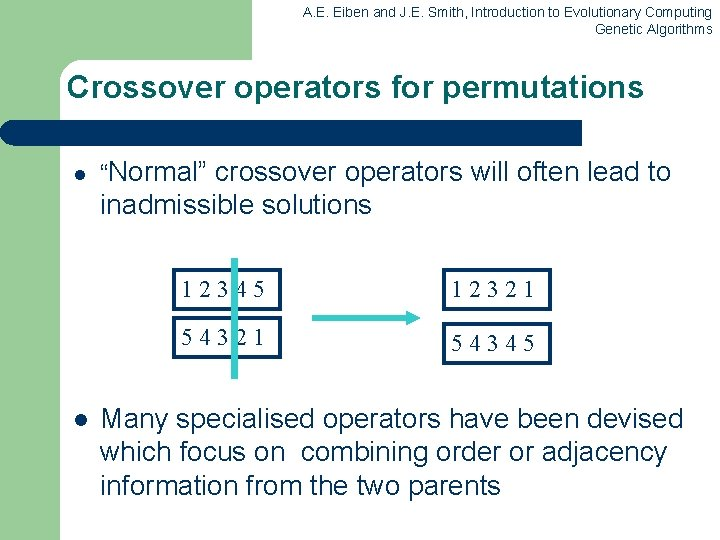A. E. Eiben and J. E. Smith, Introduction to Evolutionary Computing Genetic Algorithms Crossover operators for permutations l “Normal” crossover operators will often lead to inadmissible solutions l 12345 12321 54345 Many specialised operators have been devised which focus on combining order or adjacency information from the two parents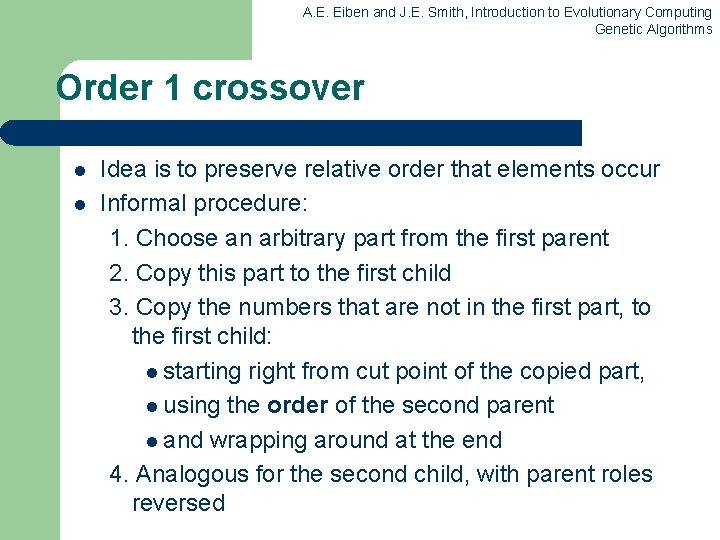A. E. Eiben and J. E. Smith, Introduction to Evolutionary Computing Genetic Algorithms Order 1 crossover l l Idea is to preserve relative order that elements occur Informal procedure: 1. Choose an arbitrary part from the first parent 2. Copy this part to the first child 3. Copy the numbers that are not in the first part, to the first child: l starting right from cut point of the copied part, l using the order of the second parent l and wrapping around at the end 4. Analogous for the second child, with parent roles reversedA. E. Eiben and J. E. Smith, Introduction to Evolutionary Computing Genetic Algorithms Order 1 crossover example l Copy randomly selected set from first parent l Copy rest from second parent in order 1, 9, 3, 8, 2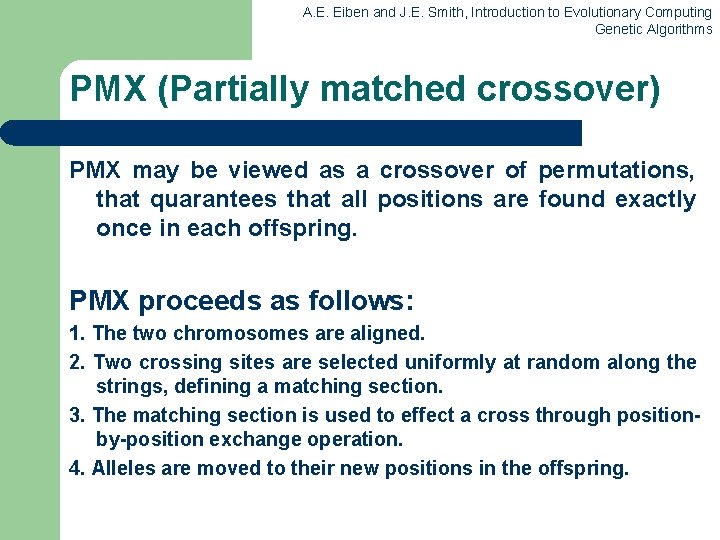A. E. Eiben and J. E. Smith, Introduction to Evolutionary Computing Genetic Algorithms PMX (Partially matched crossover) PMX may be viewed as a crossover of permutations, that quarantees that all positions are found exactly once in each offspring. PMX proceeds as follows: 1. The two chromosomes are aligned. 2. Two crossing sites are selected uniformly at random along the strings, defining a matching section. 3. The matching section is used to effect a cross through positionby-position exchange operation. 4. Alleles are moved to their new positions in the offspring.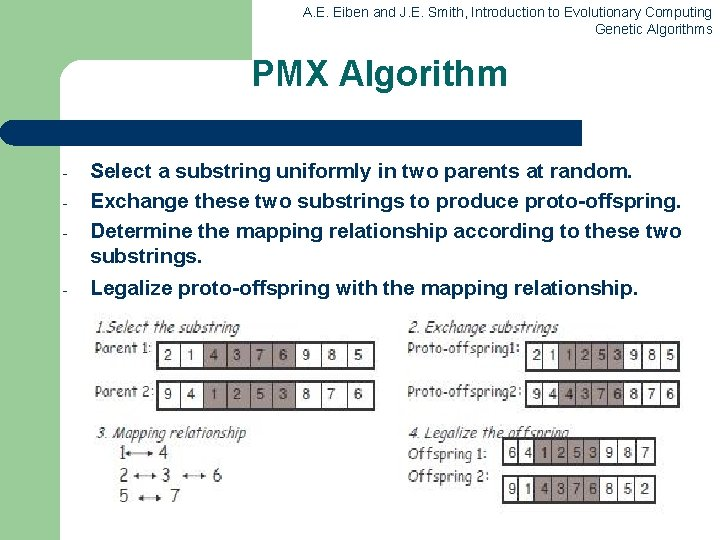A. E. Eiben and J. E. Smith, Introduction to Evolutionary Computing Genetic Algorithms PMX Algorithm - Select a substring uniformly in two parents at random. Exchange these two substrings to produce proto-offspring. Determine the mapping relationship according to these two substrings. Legalize proto-offspring with the mapping relationship.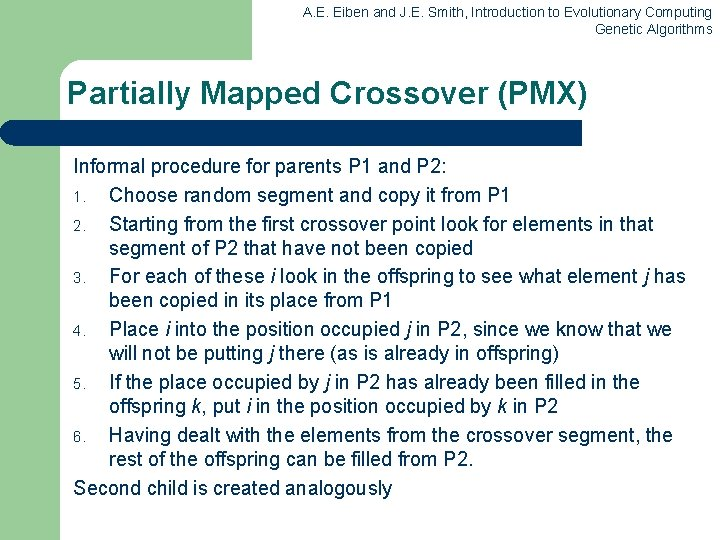A. E. Eiben and J. E. Smith, Introduction to Evolutionary Computing Genetic Algorithms Partially Mapped Crossover (PMX) Informal procedure for parents P 1 and P 2: 1. Choose random segment and copy it from P 1 2. Starting from the first crossover point look for elements in that segment of P 2 that have not been copied 3. For each of these i look in the offspring to see what element j has been copied in its place from P 1 4. Place i into the position occupied j in P 2, since we know that we will not be putting j there (as is already in offspring) 5. If the place occupied by j in P 2 has already been filled in the offspring k, put i in the position occupied by k in P 2 6. Having dealt with the elements from the crossover segment, the rest of the offspring can be filled from P 2. Second child is created analogously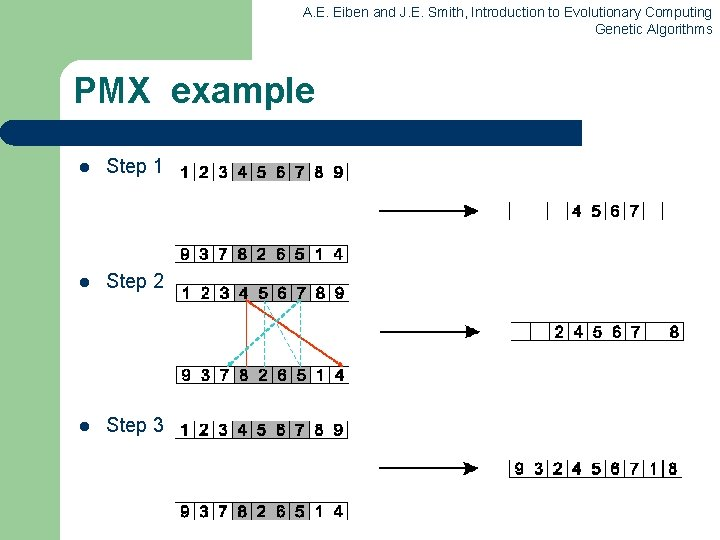A. E. Eiben and J. E. Smith, Introduction to Evolutionary Computing Genetic Algorithms PMX example l Step 1 l Step 2 l Step 3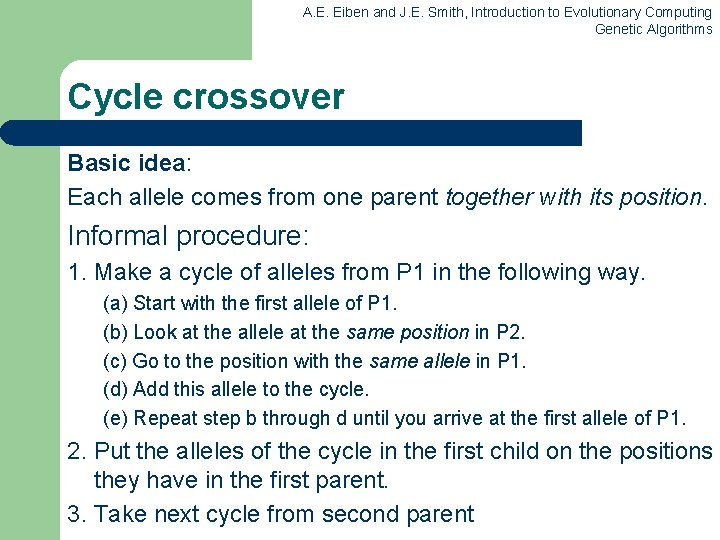A. E. Eiben and J. E. Smith, Introduction to Evolutionary Computing Genetic Algorithms Cycle crossover Basic idea: Each allele comes from one parent together with its position. Informal procedure: 1. Make a cycle of alleles from P 1 in the following way. (a) Start with the first allele of P 1. (b) Look at the allele at the same position in P 2. (c) Go to the position with the same allele in P 1. (d) Add this allele to the cycle. (e) Repeat step b through d until you arrive at the first allele of P 1. 2. Put the alleles of the cycle in the first child on the positions they have in the first parent. 3. Take next cycle from second parent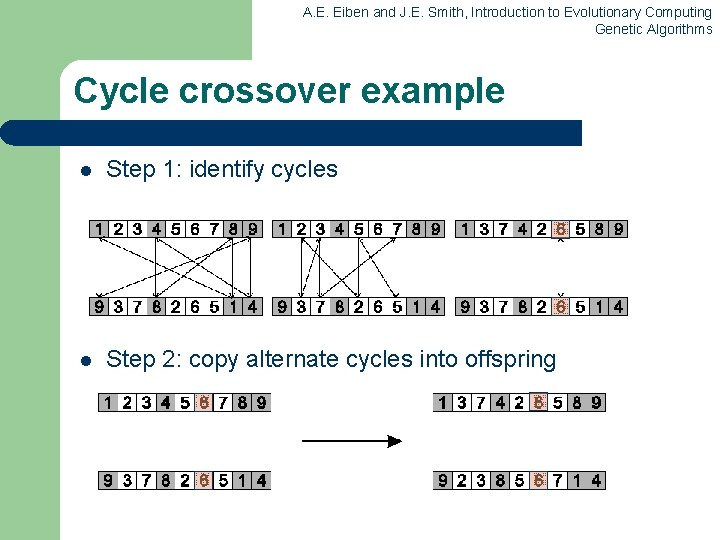A. E. Eiben and J. E. Smith, Introduction to Evolutionary Computing Genetic Algorithms Cycle crossover example l Step 1: identify cycles l Step 2: copy alternate cycles into offspring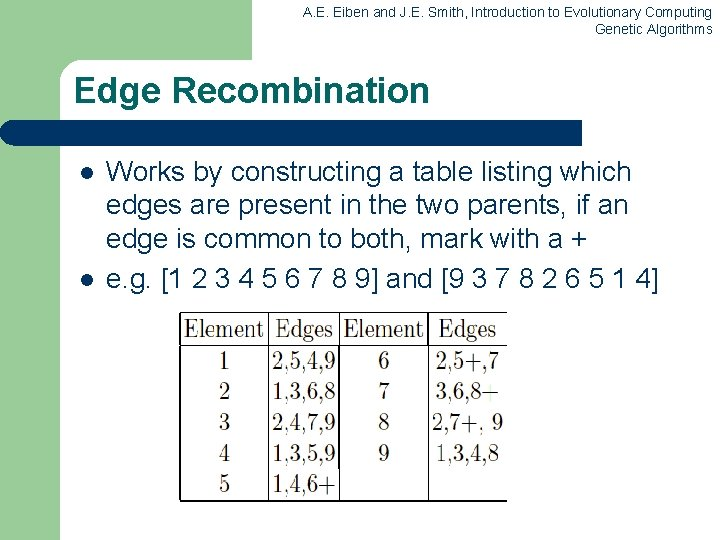A. E. Eiben and J. E. Smith, Introduction to Evolutionary Computing Genetic Algorithms Edge Recombination l l Works by constructing a table listing which edges are present in the two parents, if an edge is common to both, mark with a + e. g. [1 2 3 4 5 6 7 8 9] and [9 3 7 8 2 6 5 1 4]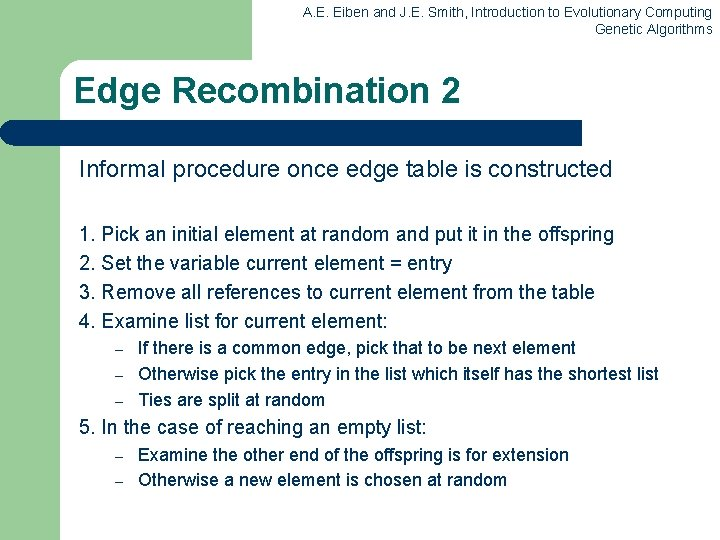A. E. Eiben and J. E. Smith, Introduction to Evolutionary Computing Genetic Algorithms Edge Recombination 2 Informal procedure once edge table is constructed 1. Pick an initial element at random and put it in the offspring 2. Set the variable current element = entry 3. Remove all references to current element from the table 4. Examine list for current element: – – – If there is a common edge, pick that to be next element Otherwise pick the entry in the list which itself has the shortest list Ties are split at random 5. In the case of reaching an empty list: – – Examine the other end of the offspring is for extension Otherwise a new element is chosen at randomA. E. Eiben and J. E. Smith, Introduction to Evolutionary Computing Genetic Algorithms Edge Recombination example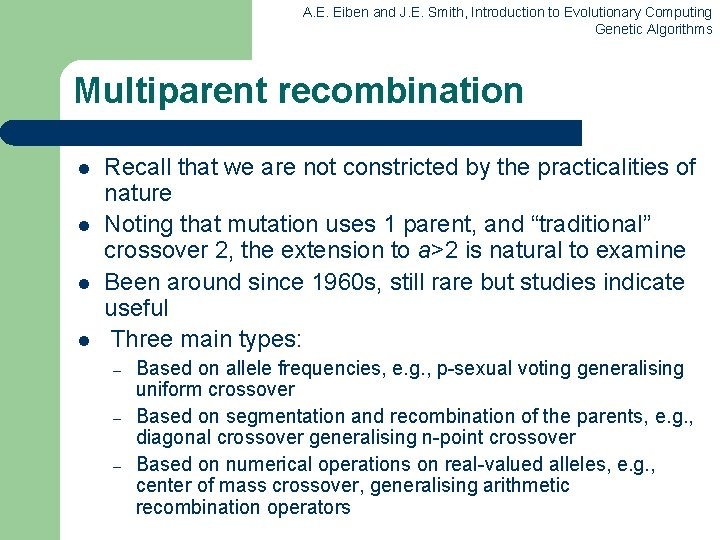A. E. Eiben and J. E. Smith, Introduction to Evolutionary Computing Genetic Algorithms Multiparent recombination l l Recall that we are not constricted by the practicalities of nature Noting that mutation uses 1 parent, and “traditional” crossover 2, the extension to a>2 is natural to examine Been around since 1960 s, still rare but studies indicate useful Three main types: – – – Based on allele frequencies, e. g. , p-sexual voting generalising uniform crossover Based on segmentation and recombination of the parents, e. g. , diagonal crossover generalising n-point crossover Based on numerical operations on real-valued alleles, e. g. , center of mass crossover, generalising arithmetic recombination operators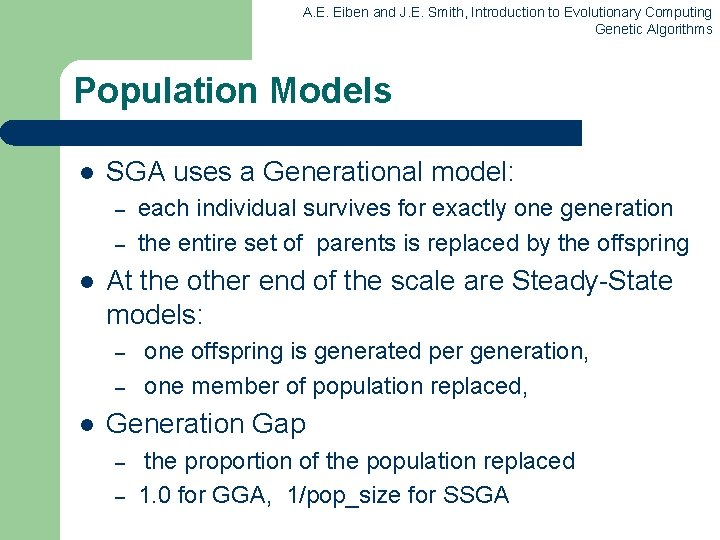A. E. Eiben and J. E. Smith, Introduction to Evolutionary Computing Genetic Algorithms Population Models l SGA uses a Generational model: – – l At the other end of the scale are Steady-State models: – – l each individual survives for exactly one generation the entire set of parents is replaced by the offspring one offspring is generated per generation, one member of population replaced, Generation Gap – – the proportion of the population replaced 1. 0 for GGA, 1/pop_size for SSGAA. E. Eiben and J. E. Smith, Introduction to Evolutionary Computing Genetic Algorithms Fitness Based Competition l Selection can occur in two places: – – l Selection operators work on whole individual – l Selection from current generation to take part in mating (parent selection) Selection from parents + offspring to go into next generation (survivor selection) i. e. they are representation-independent Distinction between selection – – operators: define selection probabilities algorithms: define how probabilities are implementedA. E. Eiben and J. E. Smith, Introduction to Evolutionary Computing Genetic Algorithms Implementation example: SGA l Expected number of copies of an individual i E(ni ) = f(i) / f ( = pop. size, f(i) = fitness of i, f avg. fitness in pop. ) f = f(i) / l Roulette wheel algorithm: – – l Given a probability distribution, spin a 1 -armed wheel n times to make n selections No guarantees on actual value of ni Baker’s SUS algorithm (Stochastic universal sampling): – n evenly spaced arms on wheel and spin once – Guarantees floor(E(n ) ) n ceil(E( n ) )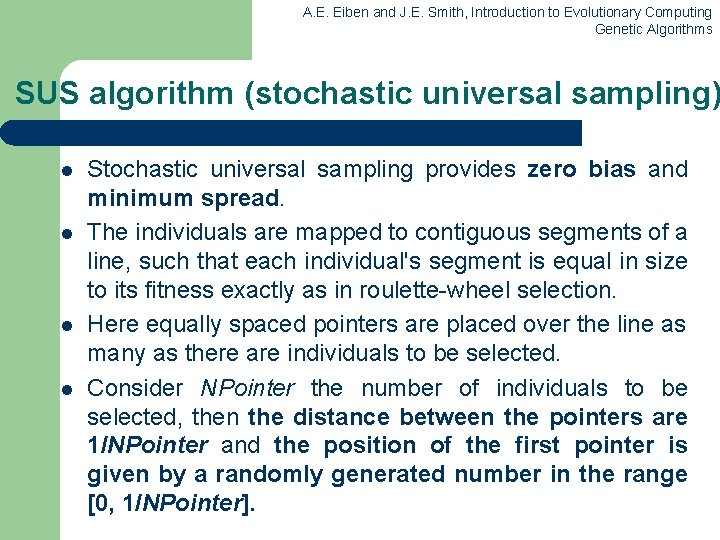A. E. Eiben and J. E. Smith, Introduction to Evolutionary Computing Genetic Algorithms SUS algorithm (stochastic universal sampling) l l Stochastic universal sampling provides zero bias and minimum spread. The individuals are mapped to contiguous segments of a line, such that each individual's segment is equal in size to its fitness exactly as in roulette-wheel selection. Here equally spaced pointers are placed over the line as many as there are individuals to be selected. Consider NPointer the number of individuals to be selected, then the distance between the pointers are 1/NPointer and the position of the first pointer is given by a randomly generated number in the range [0, 1/NPointer].A. E. Eiben and J. E. Smith, Introduction to Evolutionary Computing Genetic Algorithms SUS algorithm (continued) l l For 6 individuals to be selected, the distance between the pointers is 1/6=0. 167. Sample of 1 random number in the range [0, 0. 167]: (e. g. 0. 1) After selection the mating population consists of the individuals: 1, 2, 3, 4, 6, 8. SUS ensures a selection of offspring which is closer to what is deserved then roulette wheel selection.A. E. Eiben and J. E. Smith, Introduction to Evolutionary Computing Genetic Algorithms Fitness-Proportionate Selection l Problems include – – – l One highly fit member can rapidly take over if rest of population is much less fit: Premature Convergence At end of runs when fitnesses are similar, lose selection pressure Highly susceptible to function transposition Scaling can fix last two problems – Windowing: f’(i) = f(i) - t l – where is worst fitness in this (last n) generations Sigma Scaling: f’(i) = max( f(i) – ( f - c • f ), 0. 0) l where c is a constant, usually 2. 0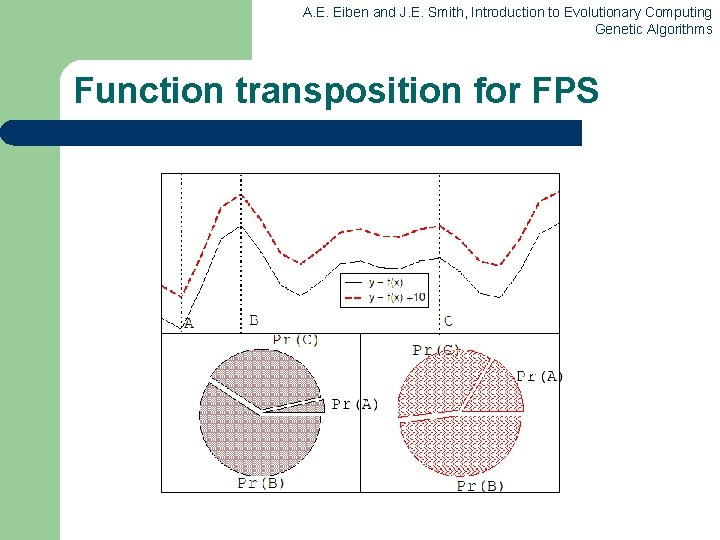A. E. Eiben and J. E. Smith, Introduction to Evolutionary Computing Genetic Algorithms Function transposition for FPSA. E. Eiben and J. E. Smith, Introduction to Evolutionary Computing Genetic Algorithms Rank – Based Selection l l l Attempt to remove problems of FPS by basing selection probabilities on relative rather than absolute fitness Rank population according to fitness and then base selection probabilities on rank where fittest has rank (or n – number of individuals) and worst rank 1 This imposes a sorting overhead on the algorithm, but this is usually negligible compared to the fitness evaluation timeA. E. Eiben and J. E. Smith, Introduction to Evolutionary Computing Genetic Algorithms Linear Ranking l l Parameterised by factor s: 1. 0 < s 2. 0 – measures advantage of best individual – ri the rank of individual i (descending sorting). – in GGA this is the number of children allotted to it Simple 3 member example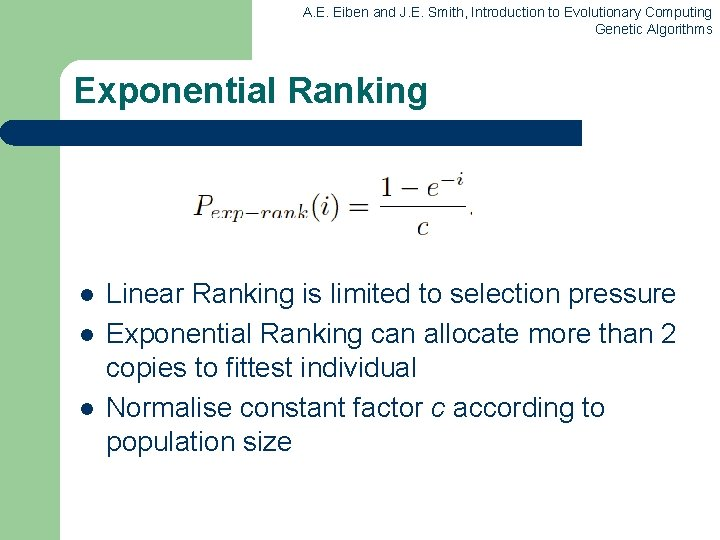A. E. Eiben and J. E. Smith, Introduction to Evolutionary Computing Genetic Algorithms Exponential Ranking l l l Linear Ranking is limited to selection pressure Exponential Ranking can allocate more than 2 copies to fittest individual Normalise constant factor c according to population size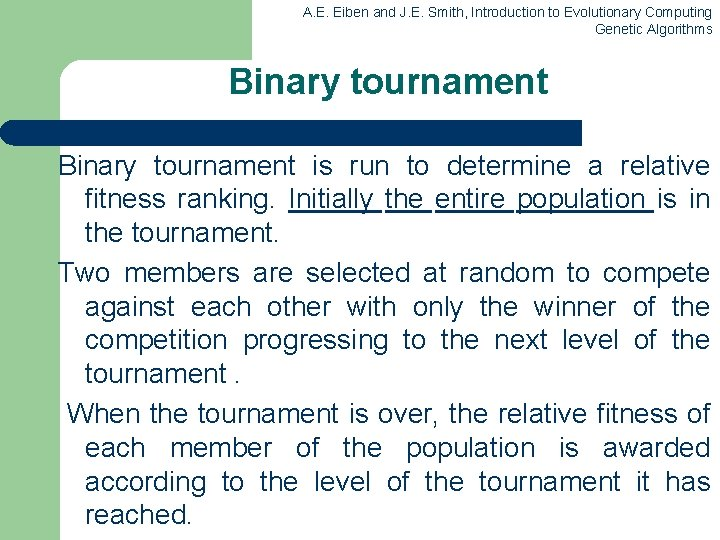A. E. Eiben and J. E. Smith, Introduction to Evolutionary Computing Genetic Algorithms Binary tournament is run to determine a relative fitness ranking. Initially the entire population is in the tournament. Two members are selected at random to compete against each other with only the winner of the competition progressing to the next level of the tournament. When the tournament is over, the relative fitness of each member of the population is awarded according to the level of the tournament it has reached.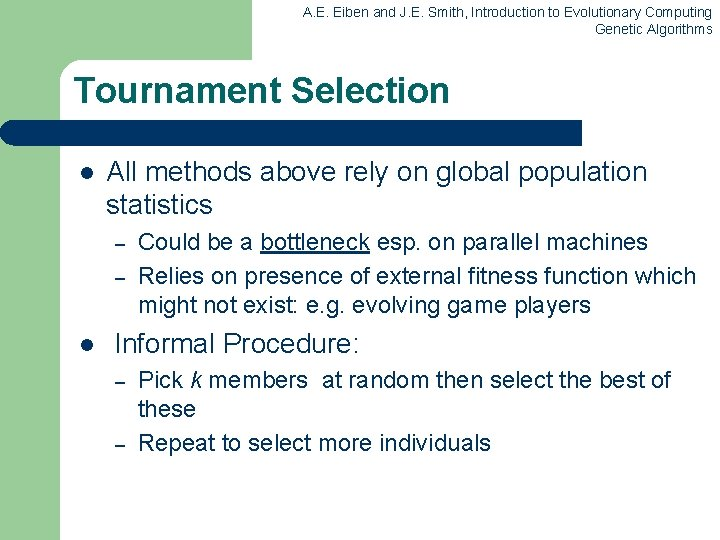A. E. Eiben and J. E. Smith, Introduction to Evolutionary Computing Genetic Algorithms Tournament Selection l All methods above rely on global population statistics – – l Could be a bottleneck esp. on parallel machines Relies on presence of external fitness function which might not exist: e. g. evolving game players Informal Procedure: – – Pick k members at random then select the best of these Repeat to select more individuals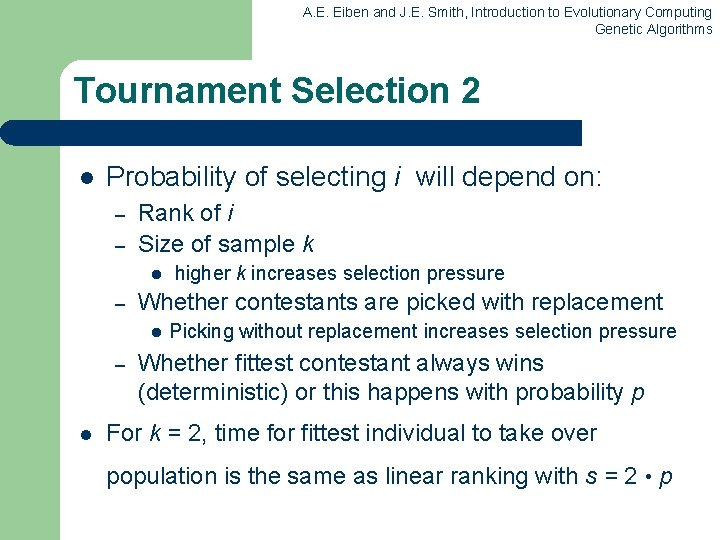A. E. Eiben and J. E. Smith, Introduction to Evolutionary Computing Genetic Algorithms Tournament Selection 2 l Probability of selecting i will depend on: – – Rank of i Size of sample k l – Whether contestants are picked with replacement l – l higher k increases selection pressure Picking without replacement increases selection pressure Whether fittest contestant always wins (deterministic) or this happens with probability p For k = 2, time for fittest individual to take over population is the same as linear ranking with s = 2 • p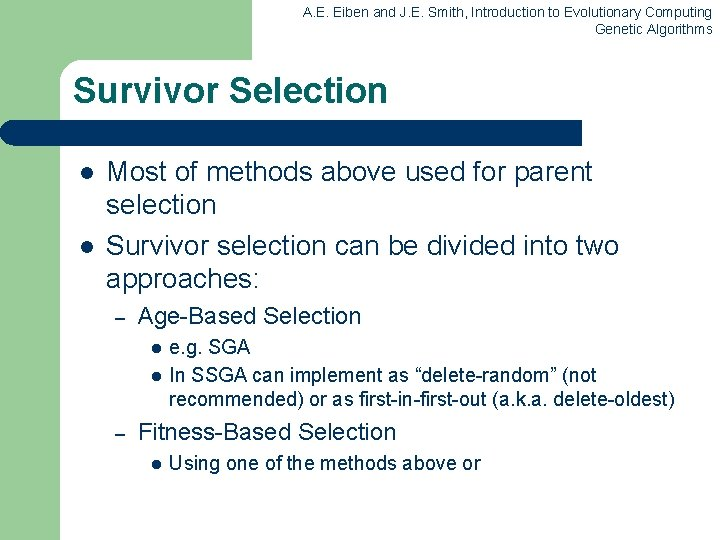A. E. Eiben and J. E. Smith, Introduction to Evolutionary Computing Genetic Algorithms Survivor Selection l l Most of methods above used for parent selection Survivor selection can be divided into two approaches: – Age-Based Selection l l – e. g. SGA In SSGA can implement as “delete-random” (not recommended) or as first-in-first-out (a. k. a. delete-oldest) Fitness-Based Selection l Using one of the methods above or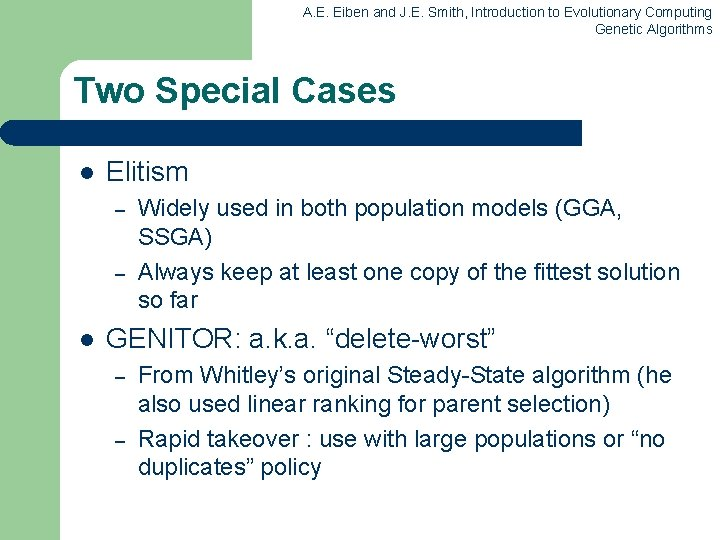A. E. Eiben and J. E. Smith, Introduction to Evolutionary Computing Genetic Algorithms Two Special Cases l Elitism – – l Widely used in both population models (GGA, SSGA) Always keep at least one copy of the fittest solution so far GENITOR: a. k. a. “delete-worst” – – From Whitley’s original Steady-State algorithm (he also used linear ranking for parent selection) Rapid takeover : use with large populations or “no duplicates” policyA. E. Eiben and J. E. Smith, Introduction to Evolutionary Computing Genetic Algorithms Example application of order based GAs: JSSP Precedence constrained job shop scheduling problem l l l J is a set of jobs. O is a set of operations M is a set of machines Able O M defines which machines can perform which operations Pre O O defines which operation should precede which Dur : O M IR defines the duration of o O on m M The goal is now to find a schedule that is: l Complete: all jobs are scheduled l Correct: all conditions defined by Able and Pre are satisfied l Optimal: the total duration of the schedule is minimalA. E. Eiben and J. E. Smith, Introduction to Evolutionary Computing Genetic Algorithms Precedence constrained job shop scheduling GA l l Representation: individuals are permutations of operations Permutations are decoded to schedules by a decoding procedure – – – take the first (next) operation from the individual look up its machine (here we assume there is only one) assign the earliest possible starting time on this machine, subject to l l l l machine occupation precedence relations holding for this operation in the schedule created so far fitness of a permutation is the duration of the corresponding schedule (to be minimized) use any suitable mutation and crossover use roulette wheel parent selection on inverse fitness Generational GA model for survivor selection use random initialisation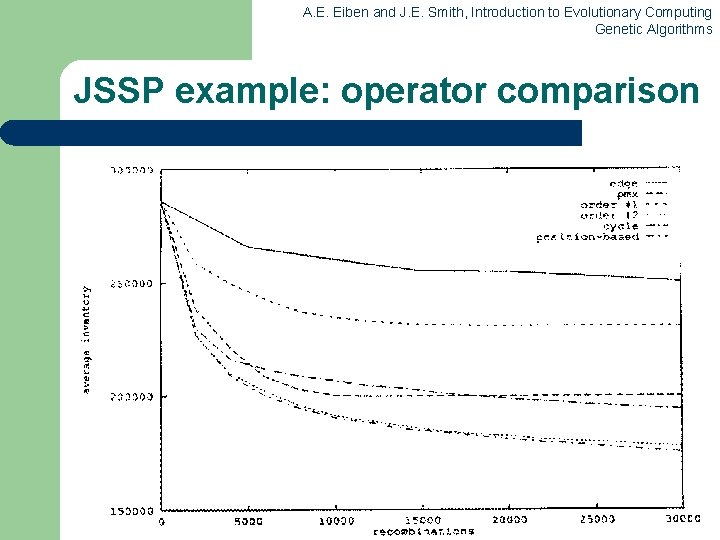A. E. Eiben and J. E. Smith, Introduction to Evolutionary Computing Genetic Algorithms JSSP example: operator comparison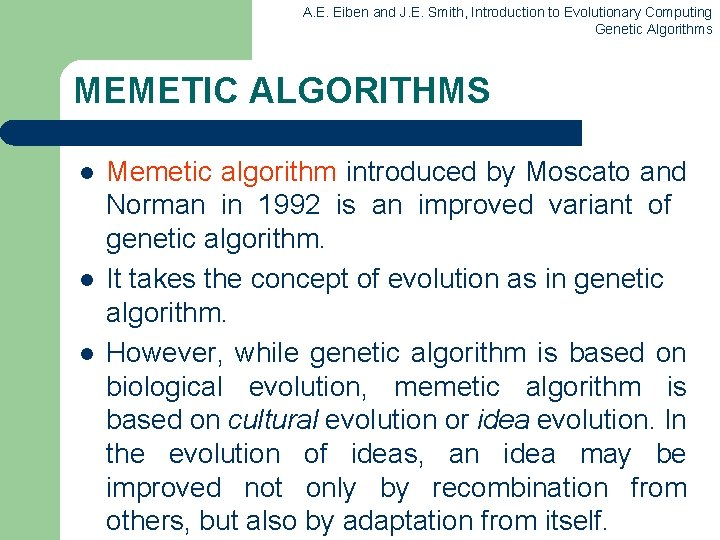A. E. Eiben and J. E. Smith, Introduction to Evolutionary Computing Genetic Algorithms MEMETIC ALGORITHMS l l l Memetic algorithm introduced by Moscato and Norman in 1992 is an improved variant of genetic algorithm. It takes the concept of evolution as in genetic algorithm. However, while genetic algorithm is based on biological evolution, memetic algorithm is based on cultural evolution or idea evolution. In the evolution of ideas, an idea may be improved not only by recombination from others, but also by adaptation from itself.A. E. Eiben and J. E. Smith, Introduction to Evolutionary Computing Genetic Algorithms A meme l l l A meme, a unit of information in memetic algorithm can be improved by the individual holding it before it is passed on. A meme differs from a gene in that as it is passed between individuals, each individual adapts the meme as it see best whereas genes are passed unchanged. A basic memetic algorithm, is then, an evolution algorithm incorporated with some local search technique.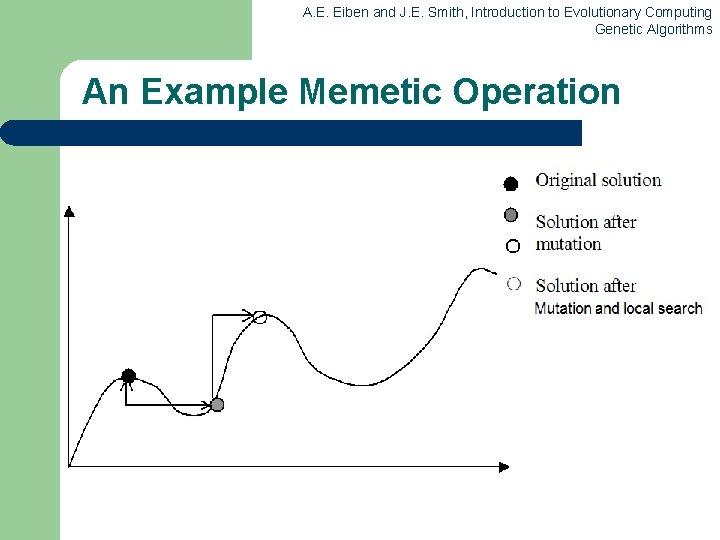A. E. Eiben and J. E. Smith, Introduction to Evolutionary Computing Genetic Algorithms An Example Memetic Operation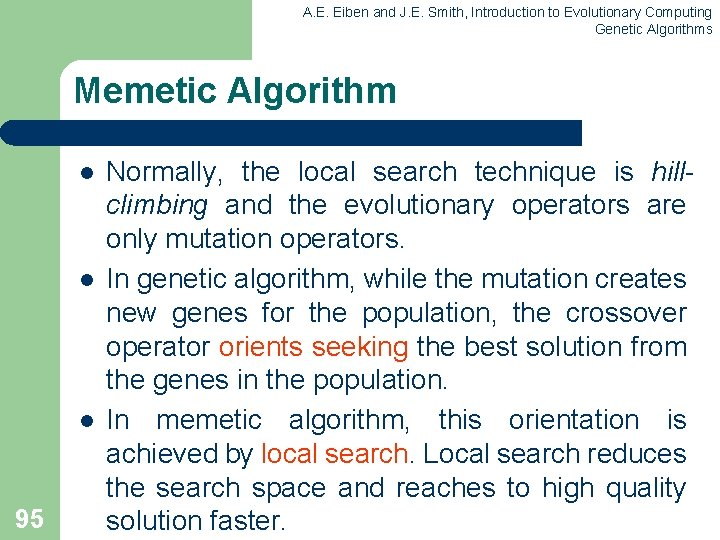A. E. Eiben and J. E. Smith, Introduction to Evolutionary Computing Genetic Algorithms Memetic Algorithm l l l 95 Normally, the local search technique is hillclimbing and the evolutionary operators are only mutation operators. In genetic algorithm, while the mutation creates new genes for the population, the crossover operator orients seeking the best solution from the genes in the population. In memetic algorithm, this orientation is achieved by local search. Local search reduces the search space and reaches to high quality solution faster.A. E. Eiben and J. E. Smith, Introduction to Evolutionary Computing Genetic Algorithms Optimized Simulated Annealing for Network-on-Chip Application Mapping l l l Simulating Annealing (SA) is a tree search technique that combines hill climbing with a random walk in order to yield efficiency and also completeness. The hill climbing algorithm never makes downhill moves so it is guaranteed to be incomplete (because it can get stuck in a local maximum). On the other hand, moving to a successor tree node, chosen randomly from all the possible successors, is very inefficient but more complete. Simulated Annealing reduces to Hill Climbing. The idea behind this algorithm comes from metallurgy, where annealing is the process used to temper or harden metals and glass by heating them to a high temperature and then gradually cooling them, thus allowing the material to coalesce into a low-energy crystalline state. Using Optimized Simulated Annealing algorithm as a mutation operator it obtain a hybrid algorithm: an Evolutionary Algorithm which incorporates a Simulated Annealing technique [Ciprian Radu]. OSA performs a context-aware mapping and outputs two cores which must be swapped. Using domain-knowledge, the Optimized Simulated Annealing (OSA) algorithm performs a dynamic and implicit core clustering and limits the number of iterations per annealing temperature based on the given application and network.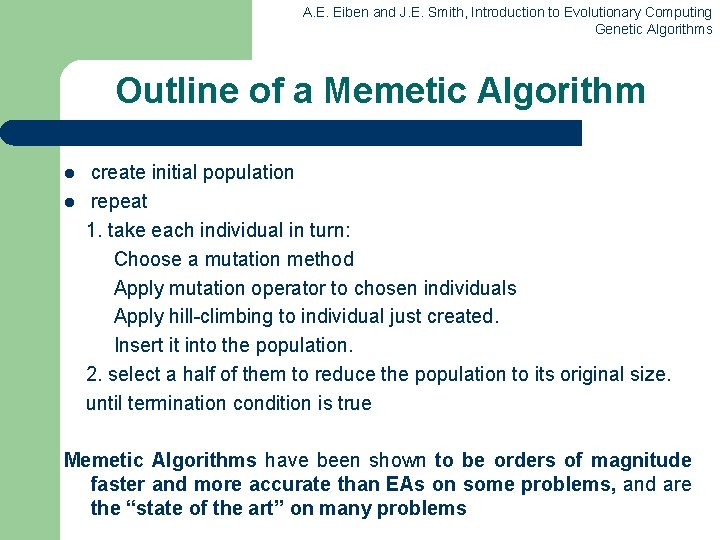A. E. Eiben and J. E. Smith, Introduction to Evolutionary Computing Genetic Algorithms Outline of a Memetic Algorithm l l create initial population repeat 1. take each individual in turn: Choose a mutation method Apply mutation operator to chosen individuals Apply hill-climbing to individual just created. Insert it into the population. 2. select a half of them to reduce the population to its original size. until termination condition is true Memetic Algorithms have been shown to be orders of magnitude faster and more accurate than EAs on some problems, and are the “state of the art” on many problems